# ML Aggarwal Solutions for Class 8 Maths Chapter 13 Understanding Quadrilaterals

ML Aggarwal Solutions for Class 8 Maths Chapter 13 Understanding Quadrilaterals are available here. Our expert tutors formulate these exercises to assist you with your exam preparation to attain good marks in Maths. Students who wish to score good marks in Maths diligently practise ML Aggarwal Solutions. Scoring high marks requires a good amount of practice on every topic.

Chapter 13 – Understanding Quadrilaterals. A quadrilateral is a polygon with four edges (or sides) and four vertices or corners, like a square, rectangle, or rhombus. In this ML Aggarwal Solutions for Class 8 Maths Chapter 13, students are going to learn finding angles and sides of quadrilaterals and the properties of quadrilaterals.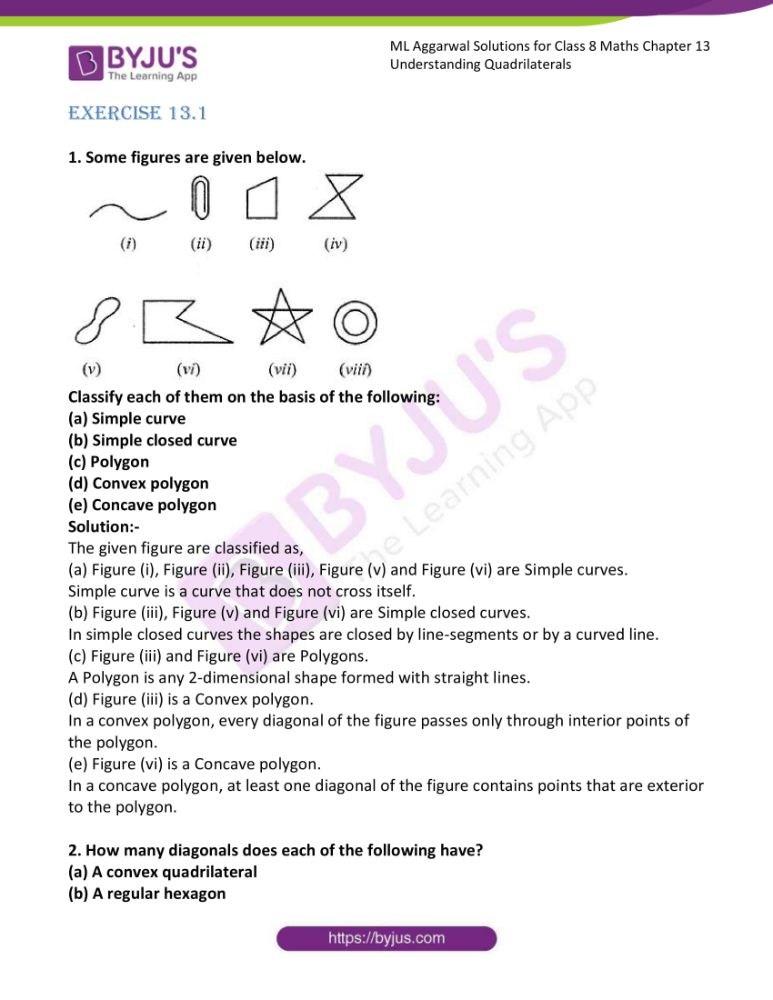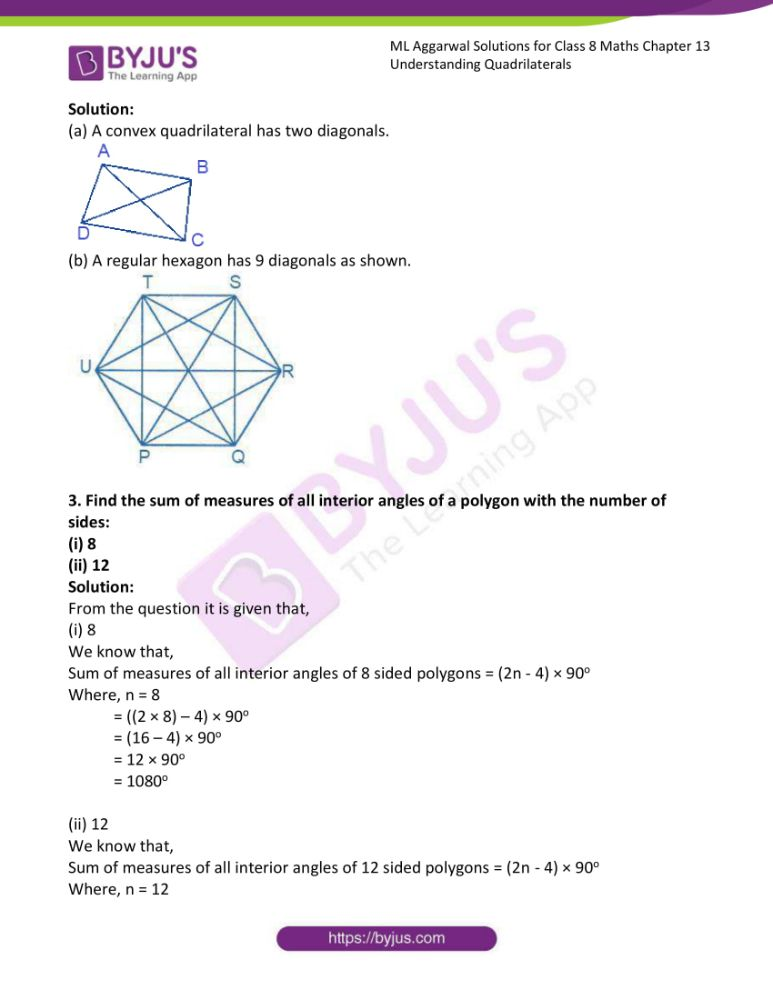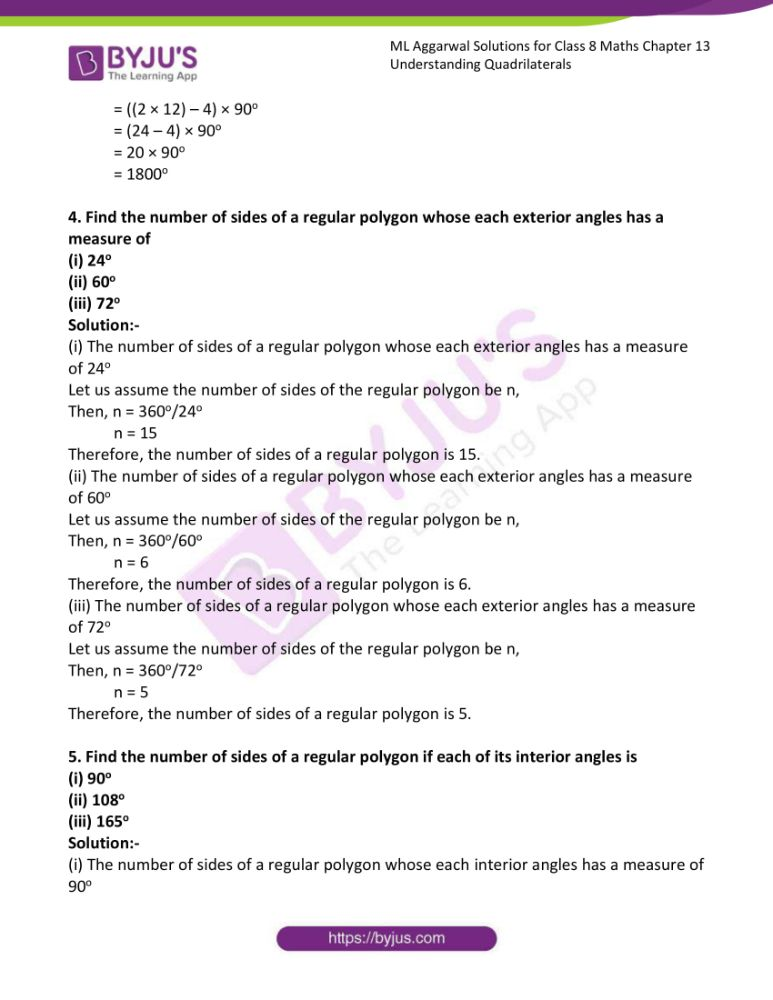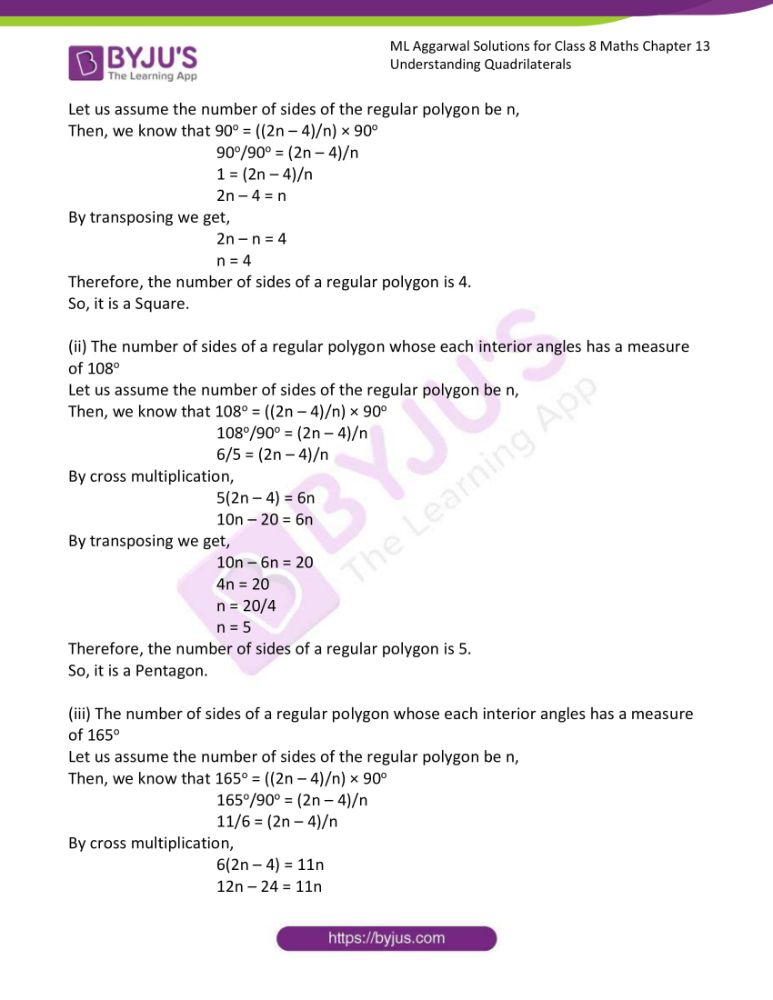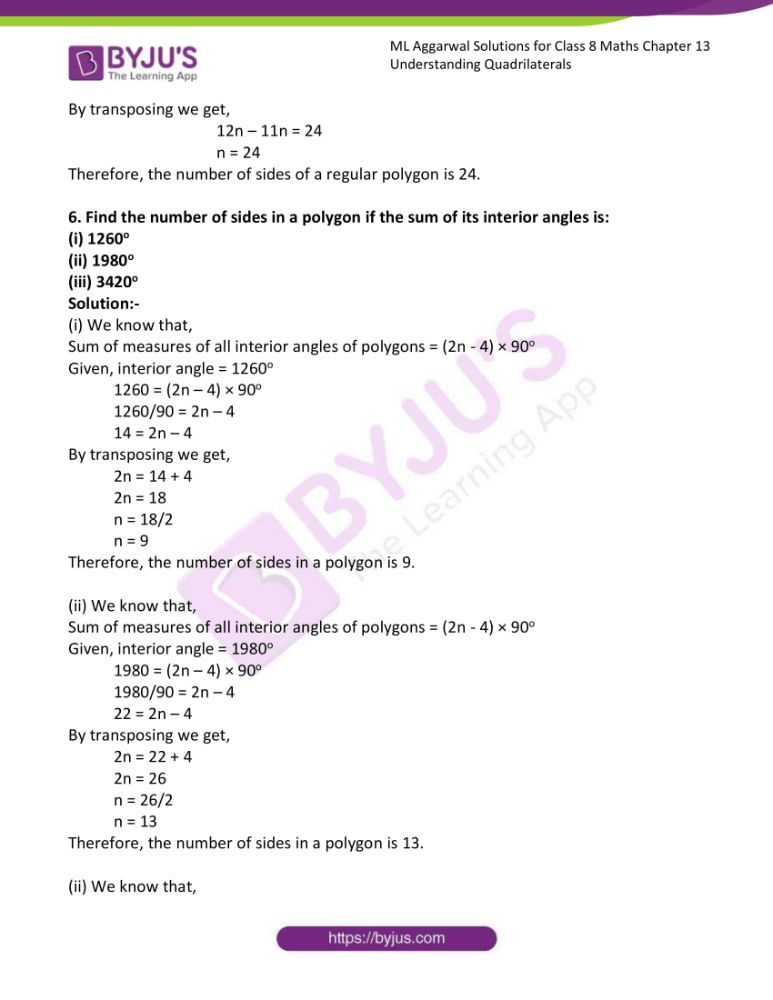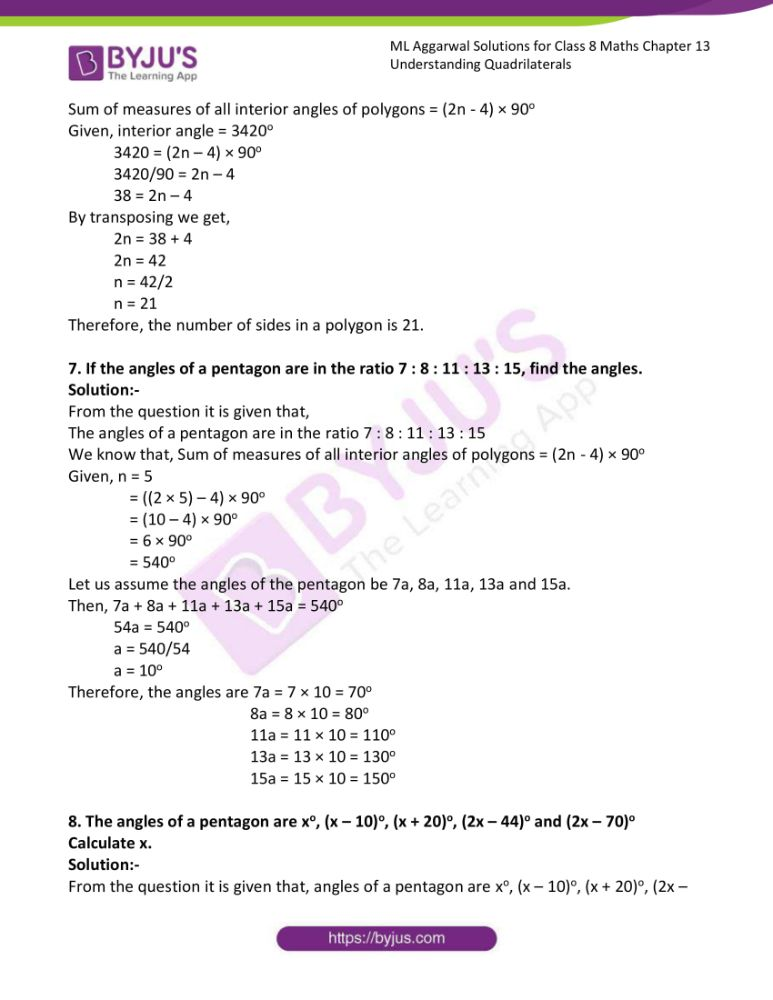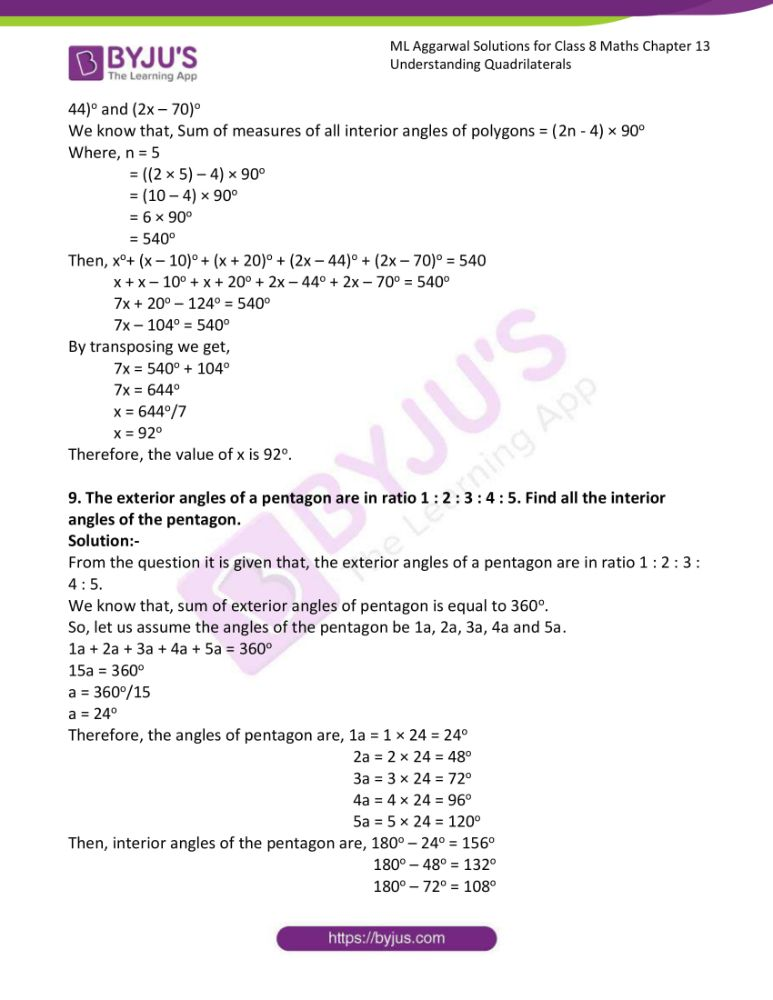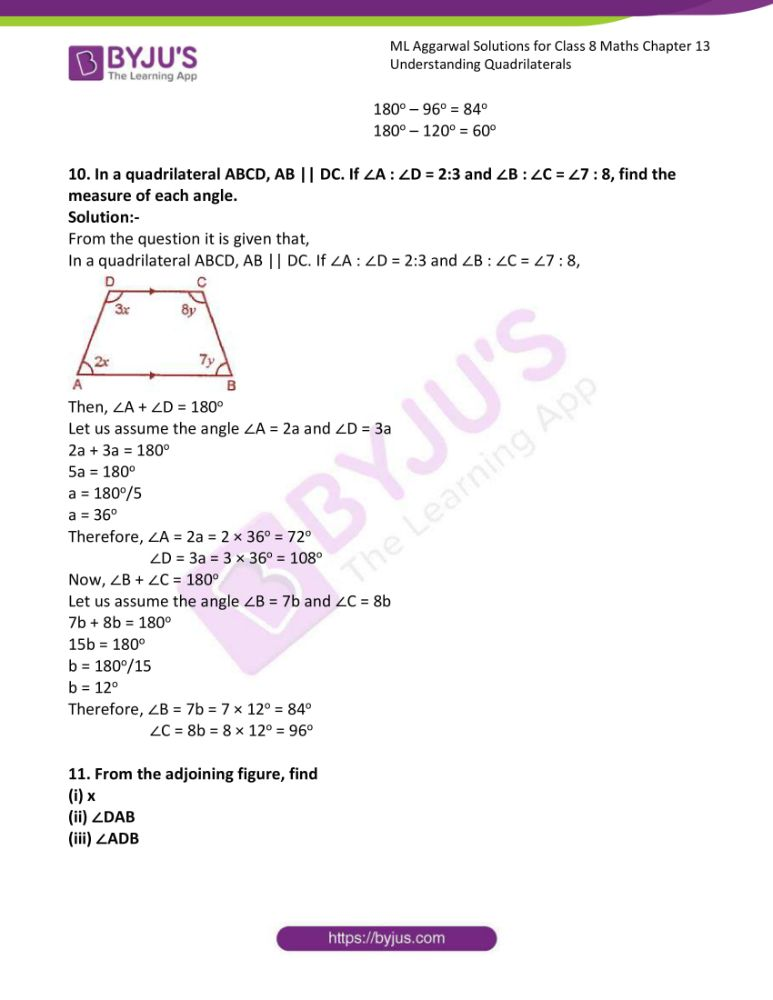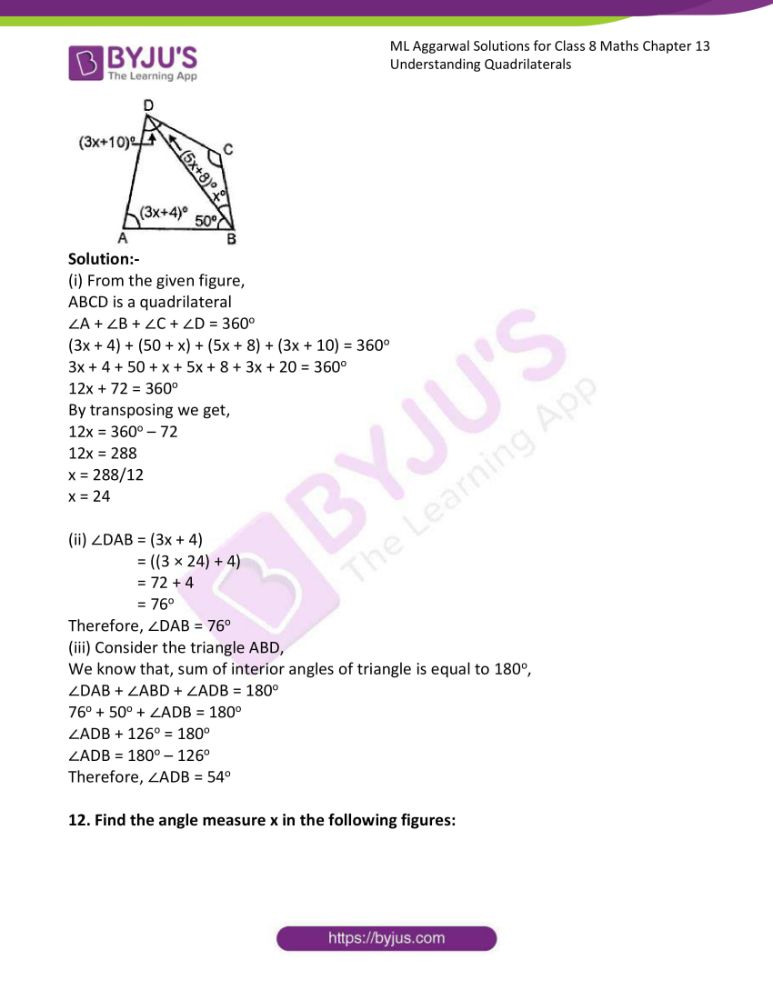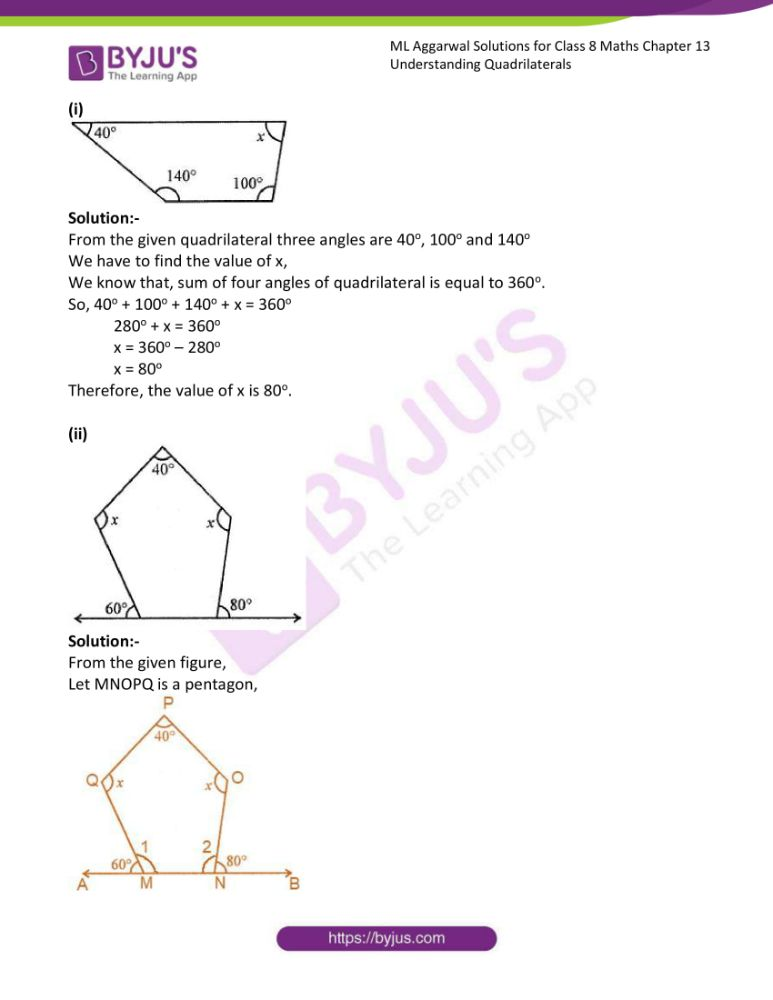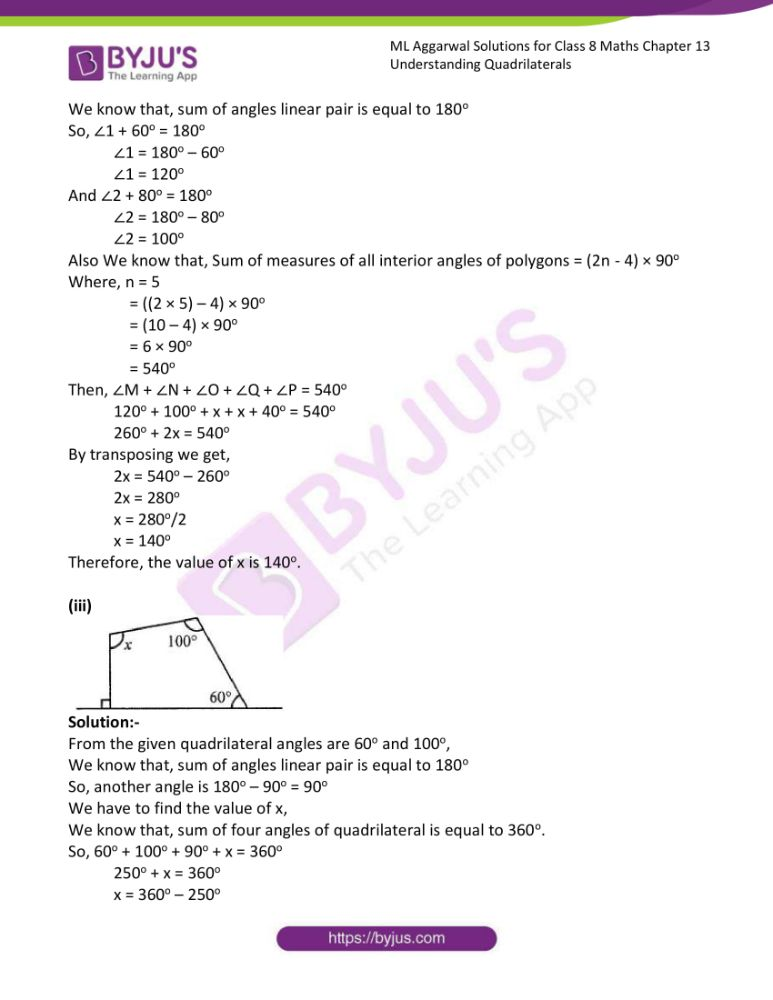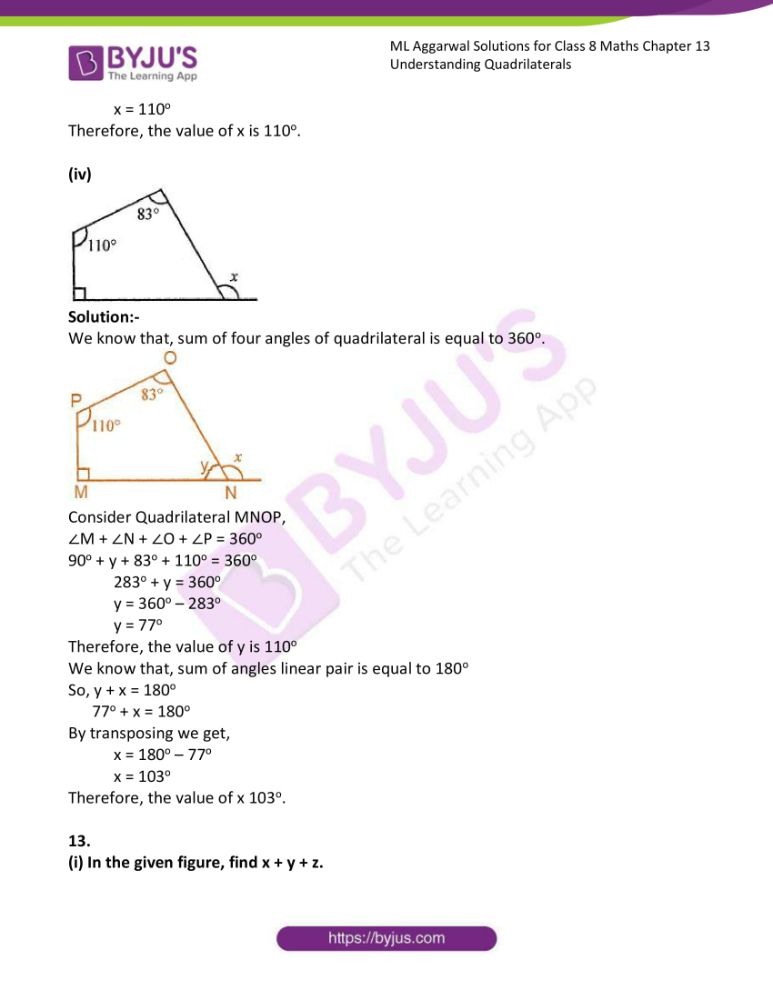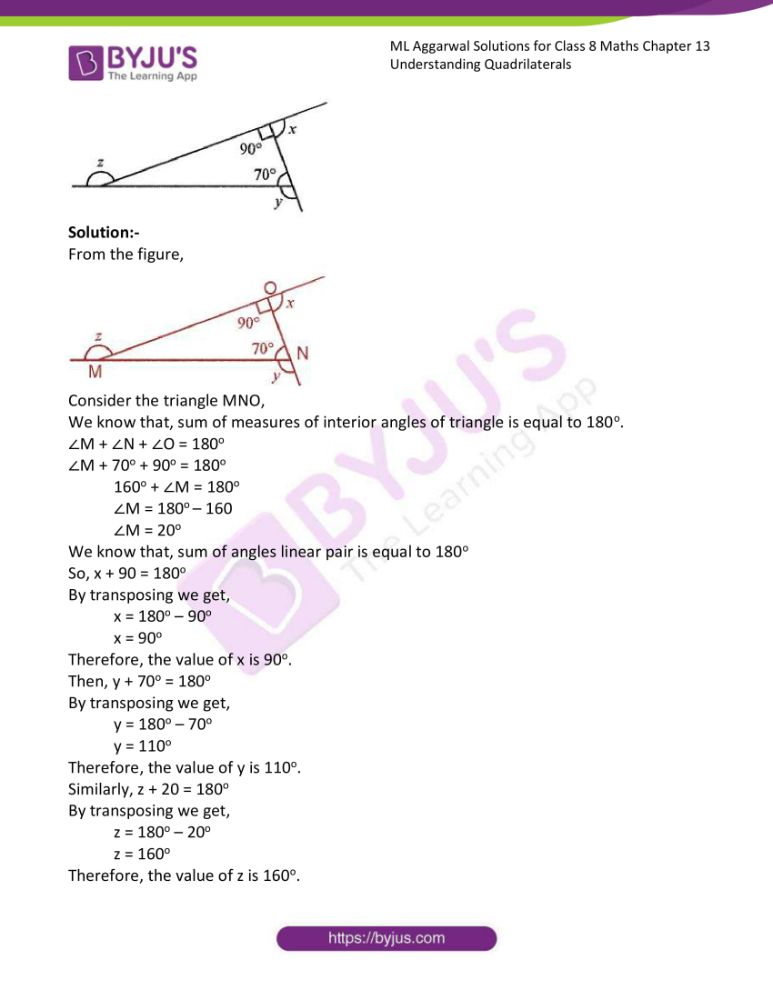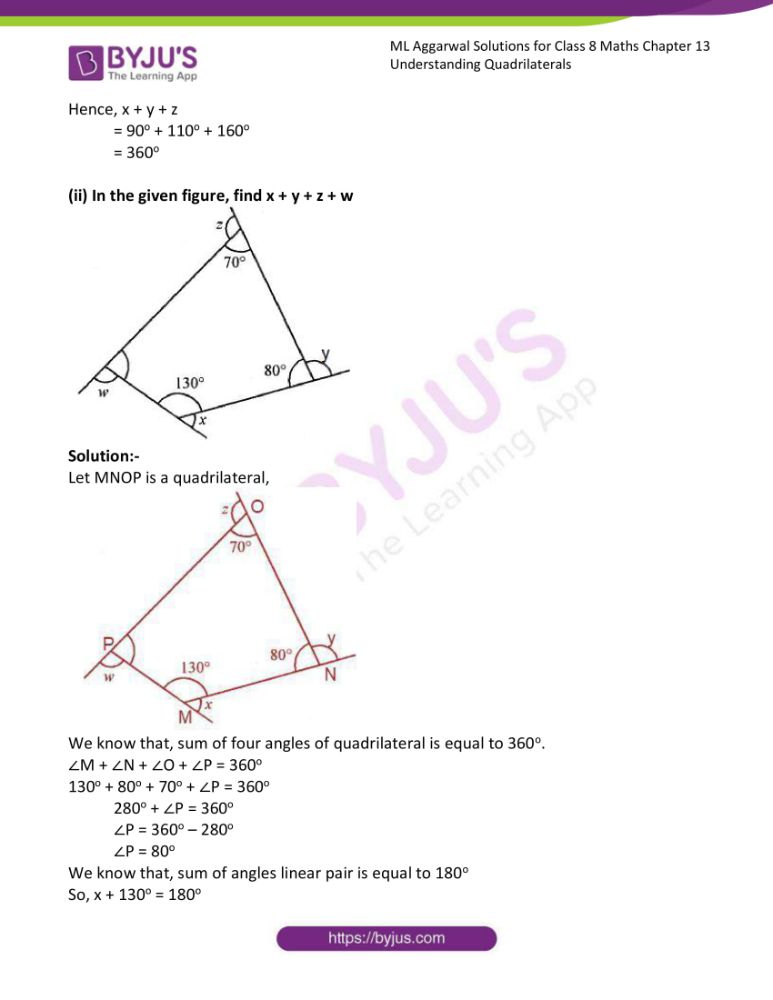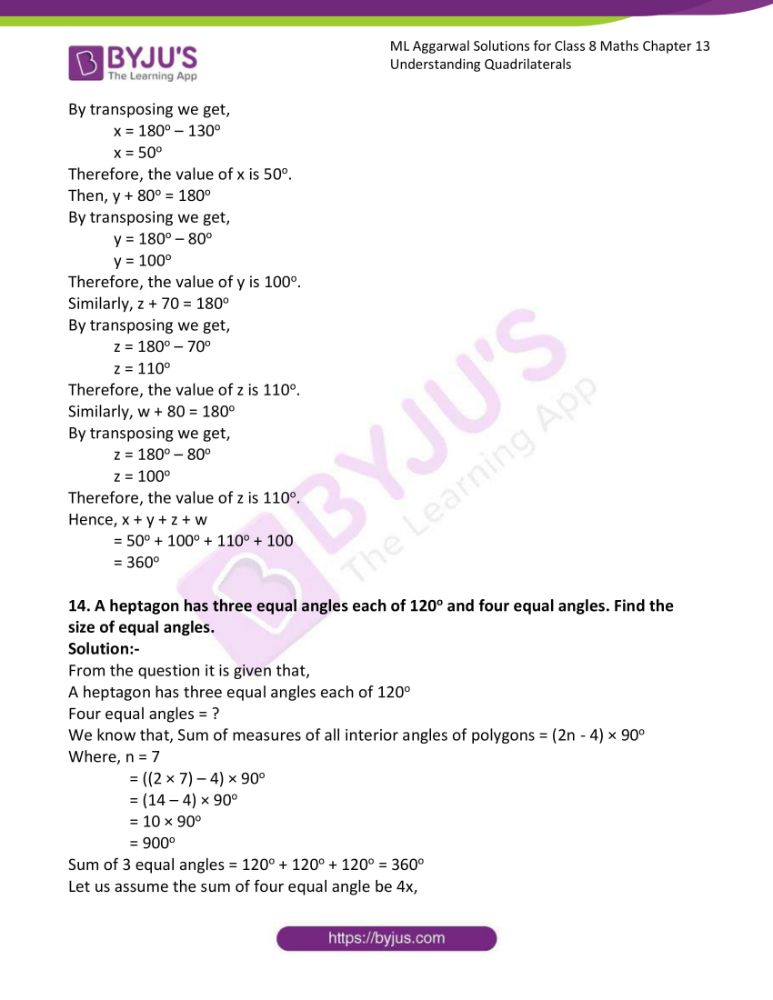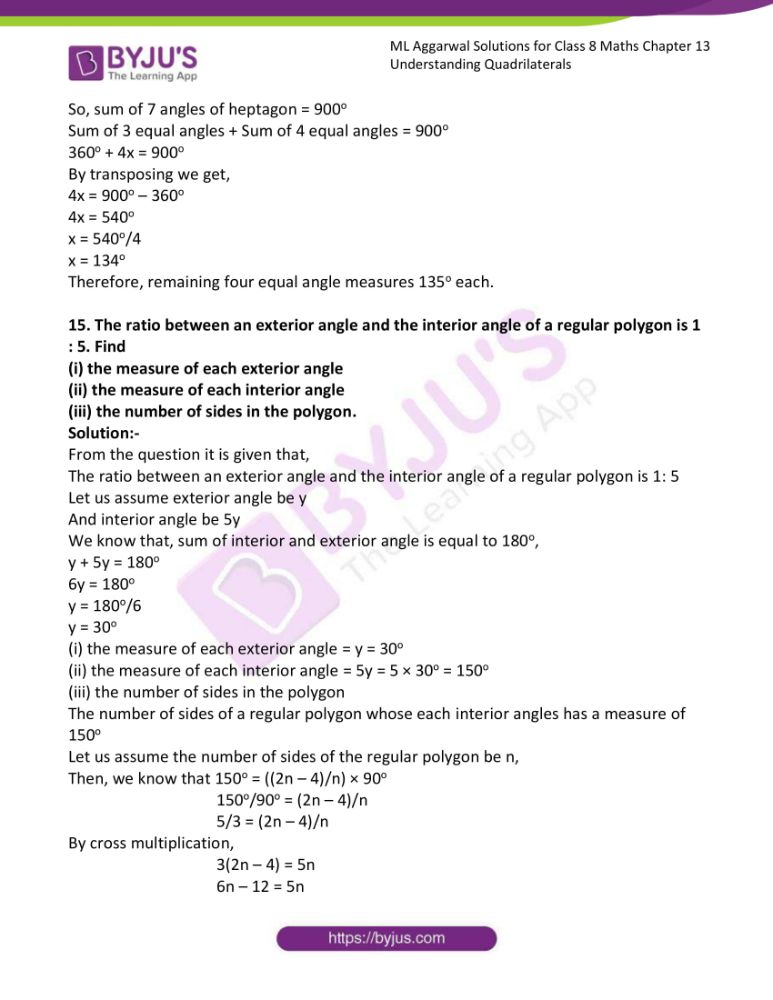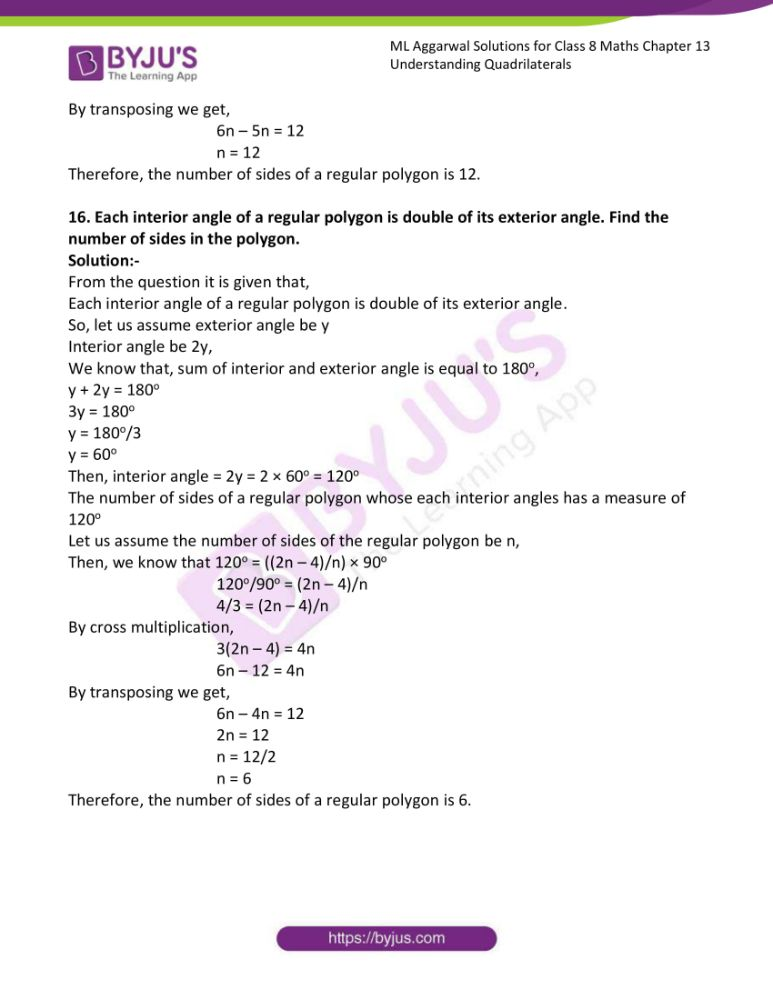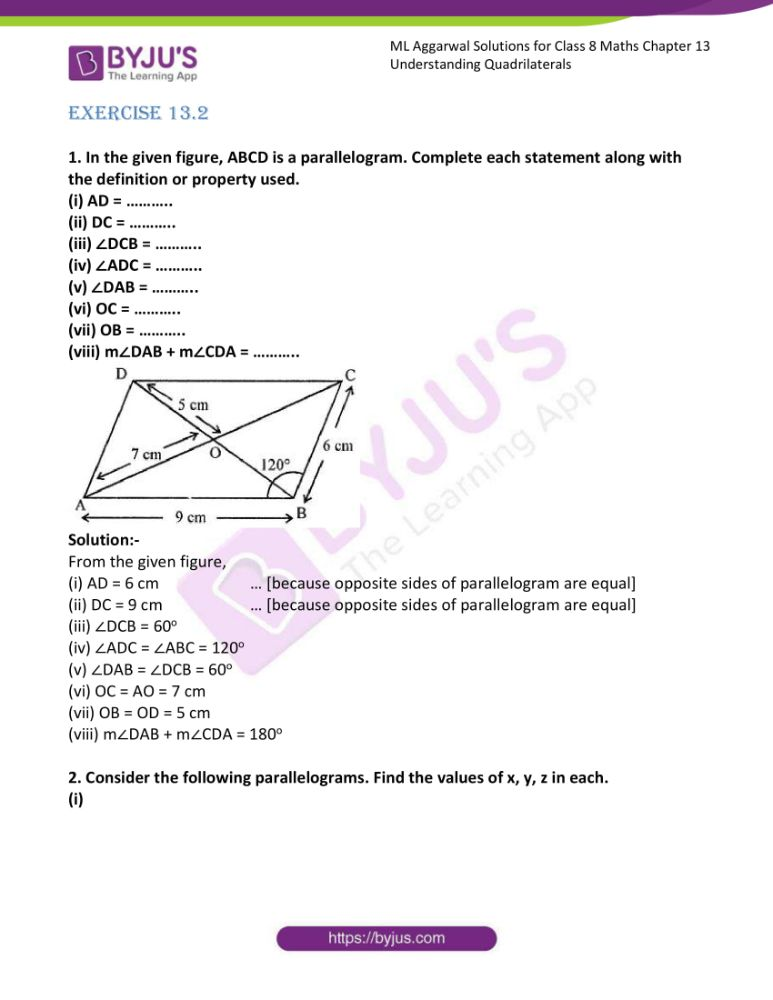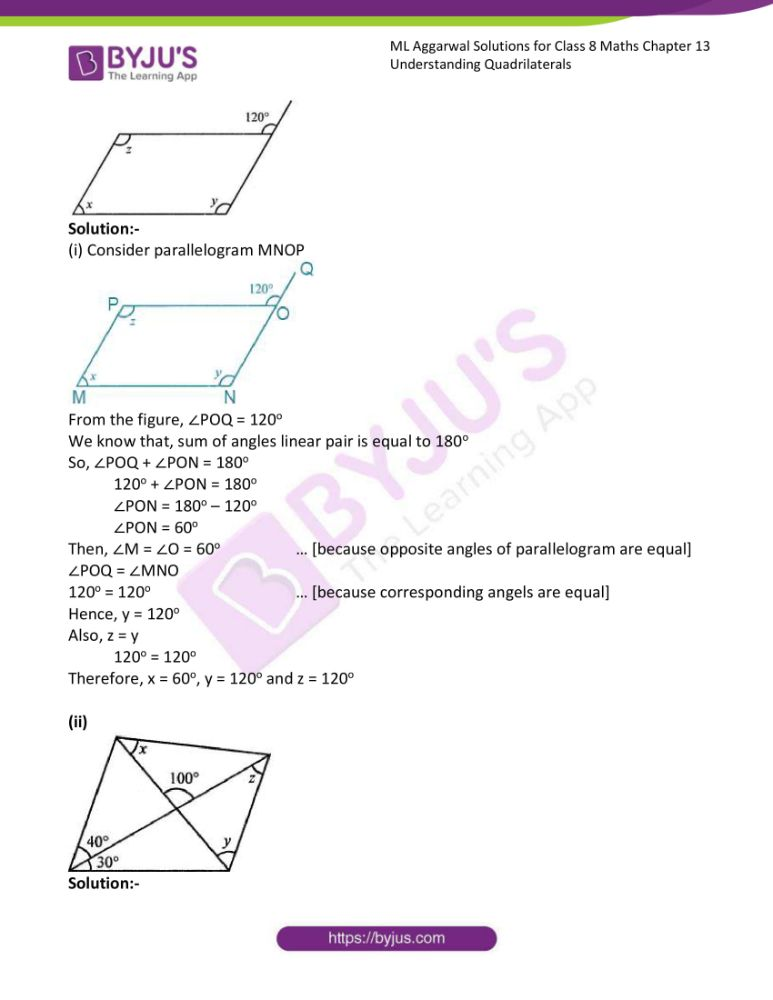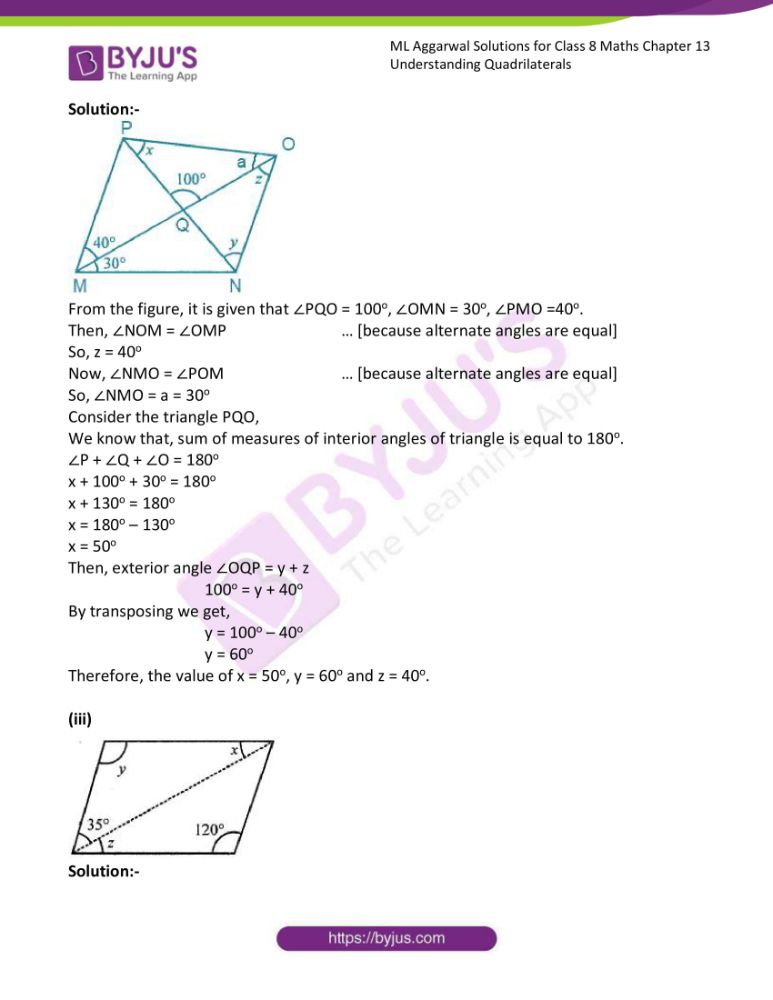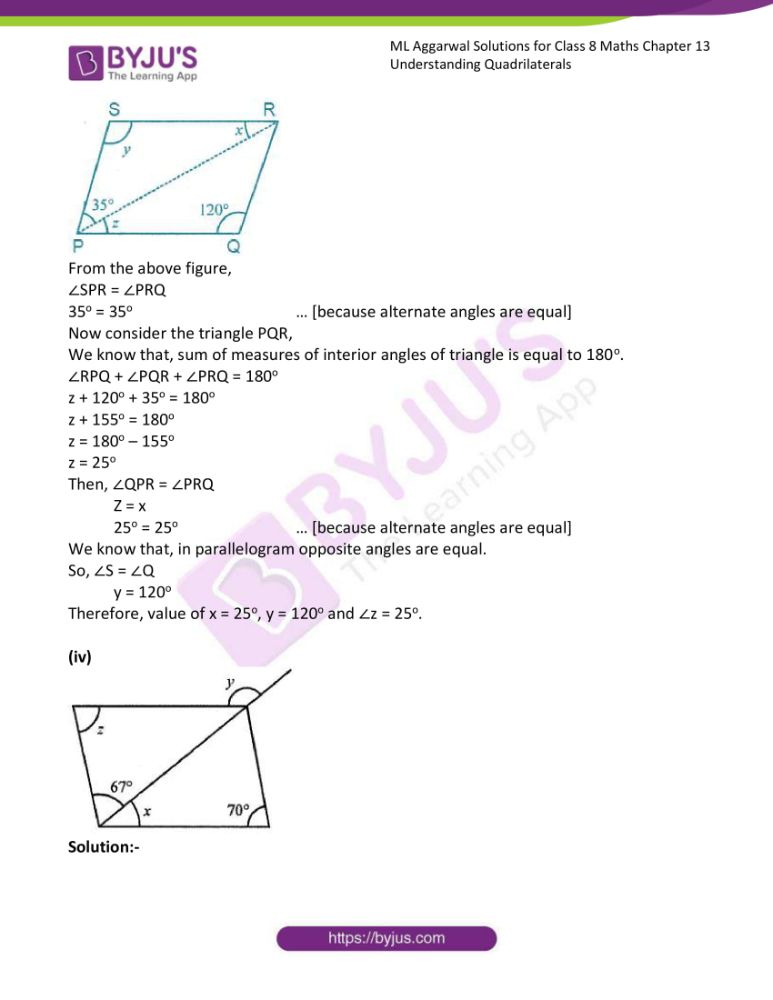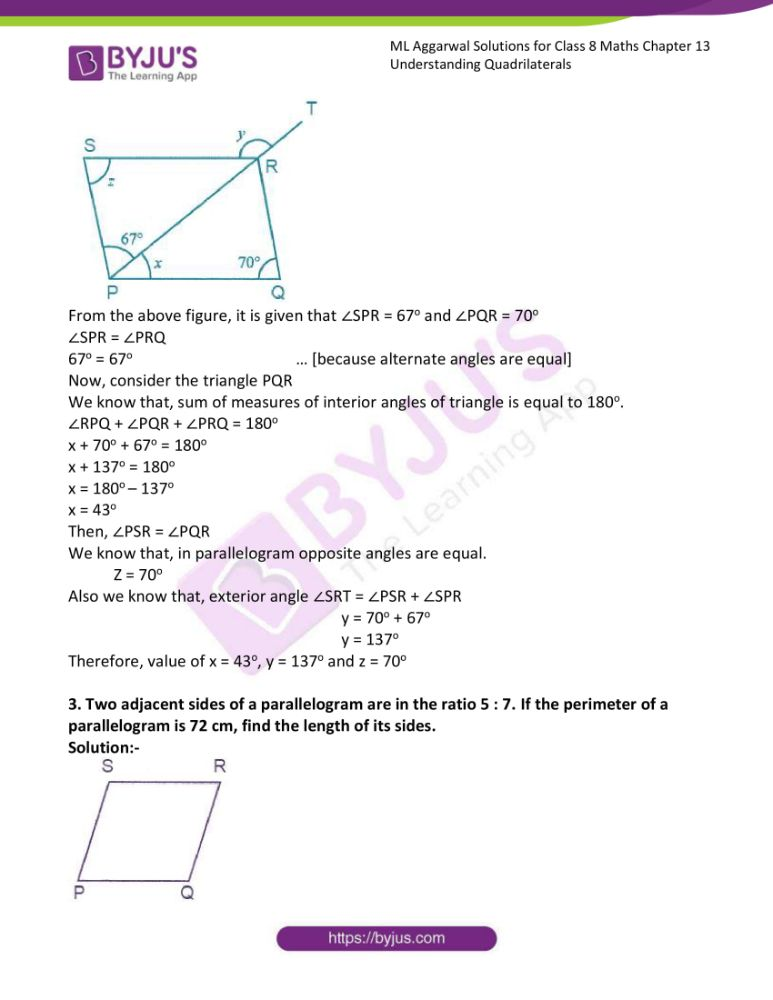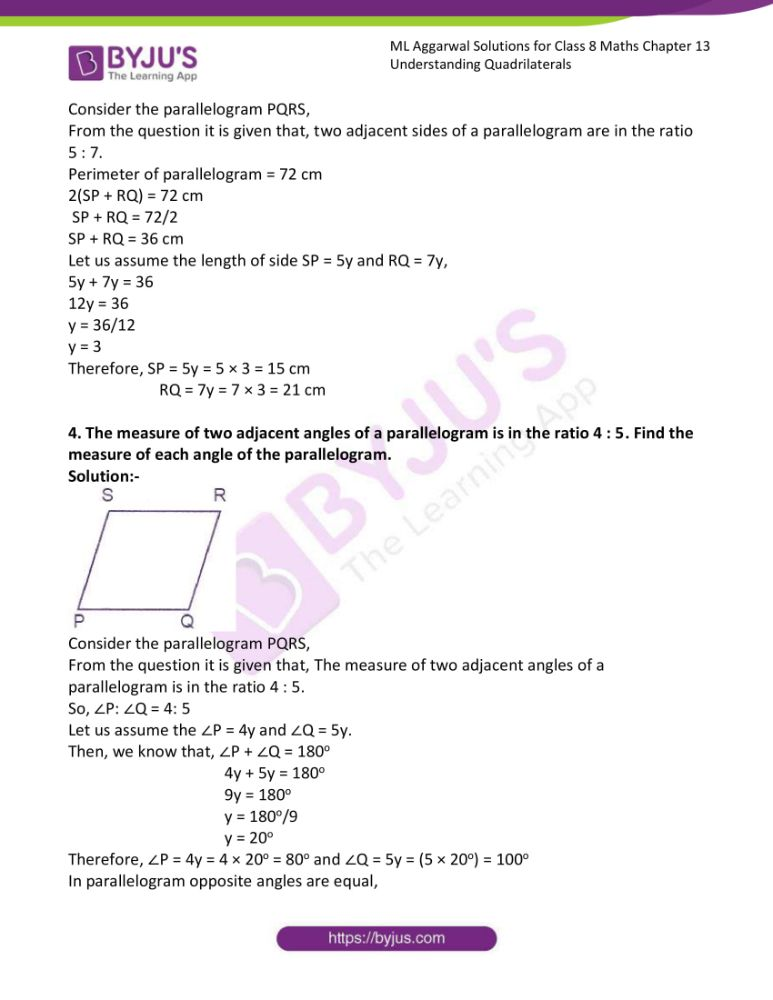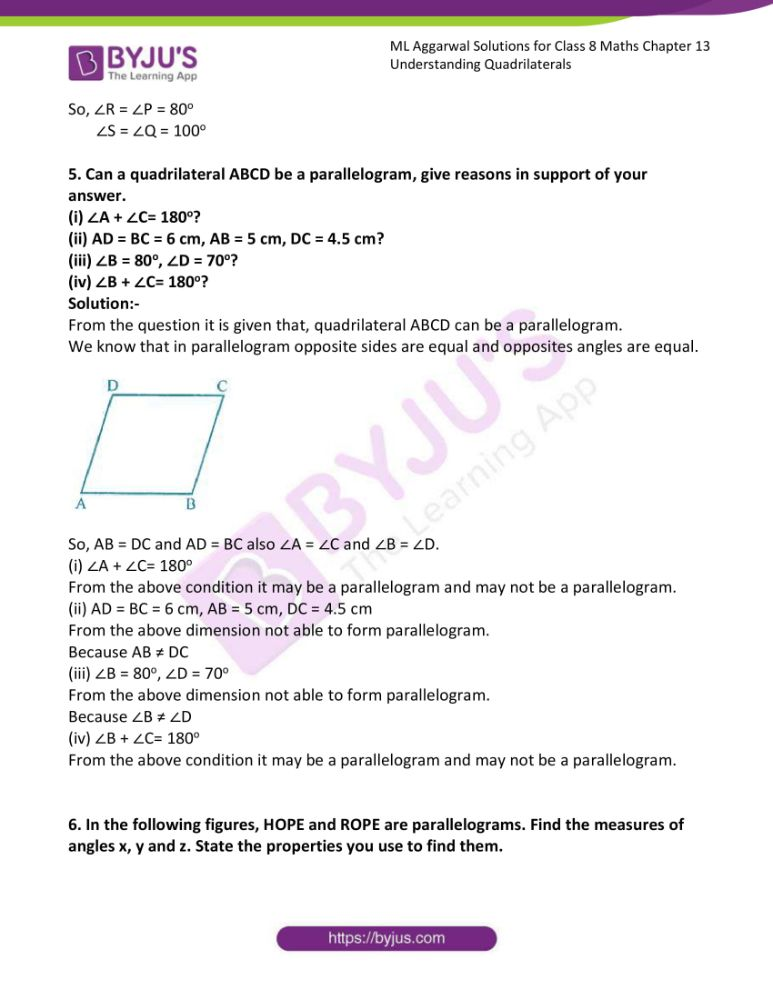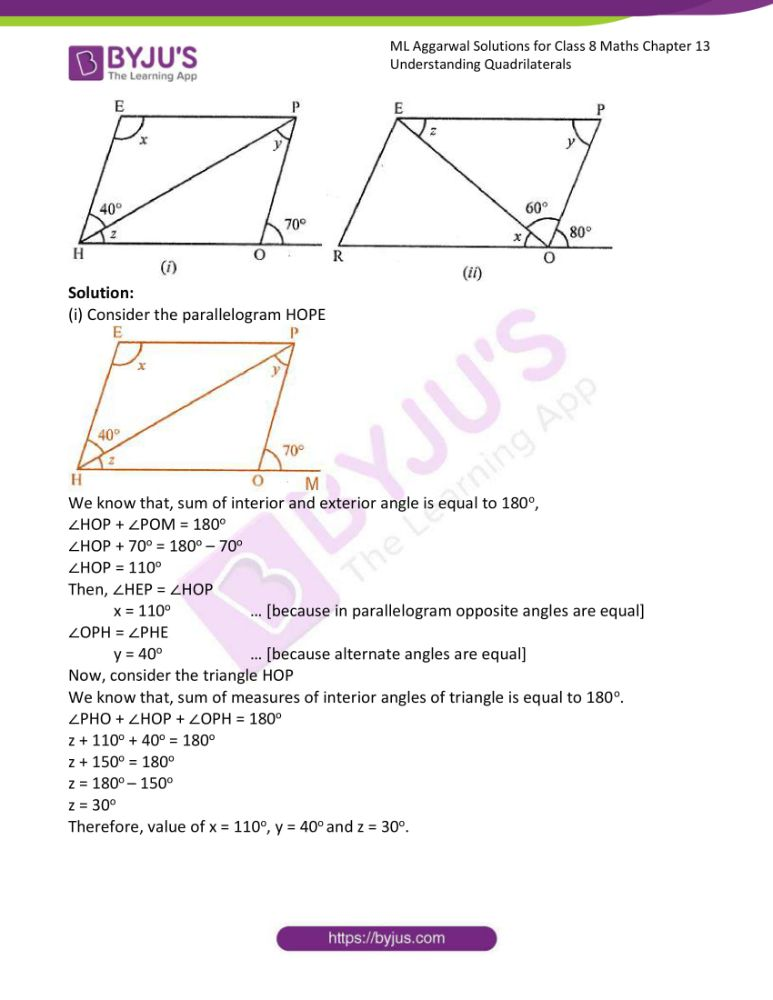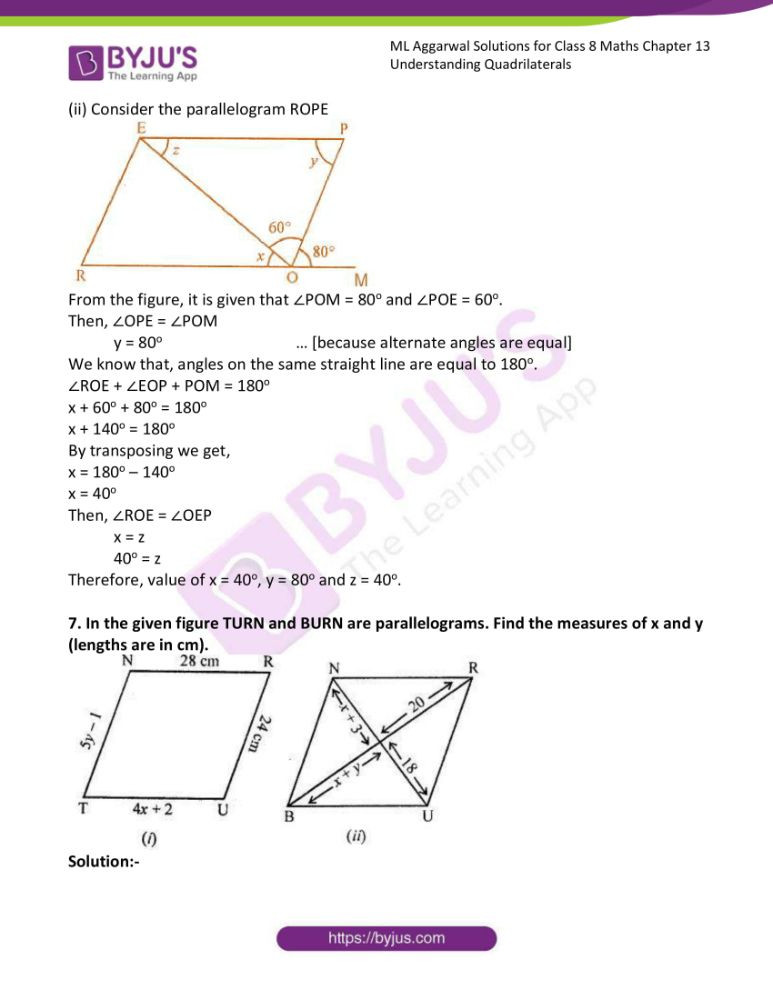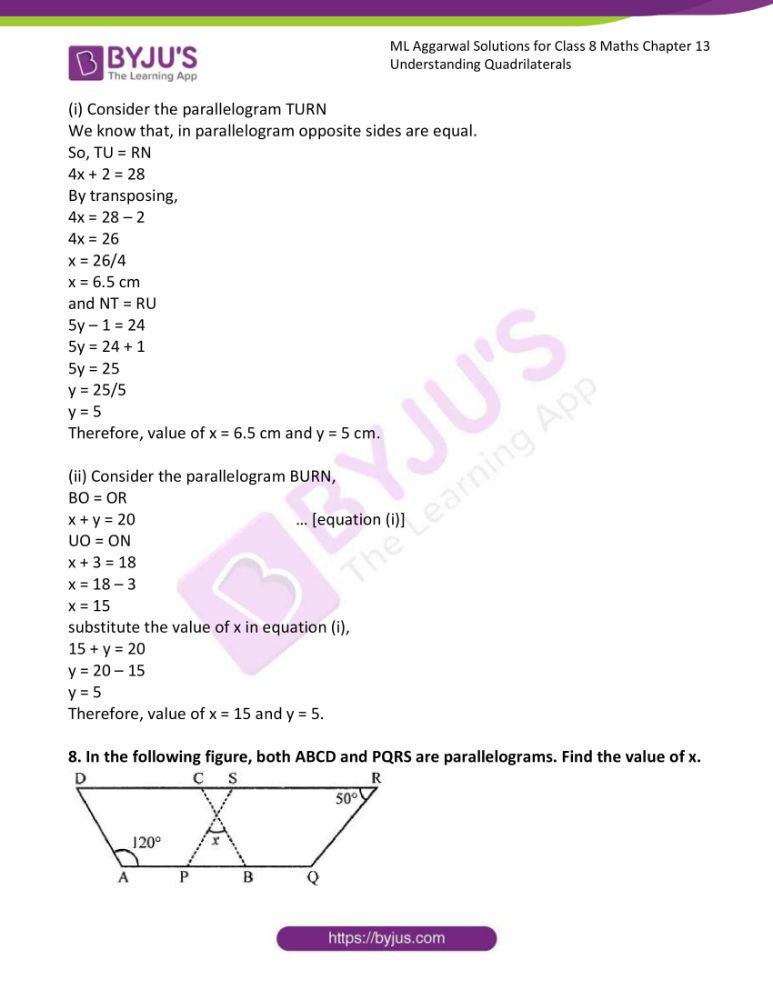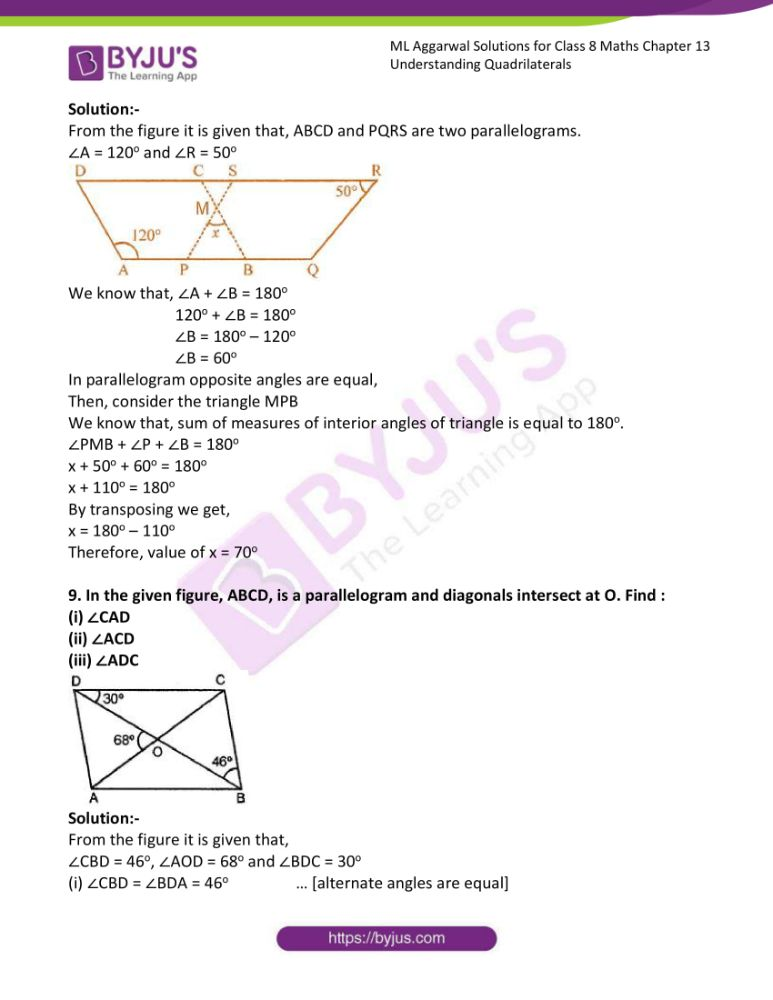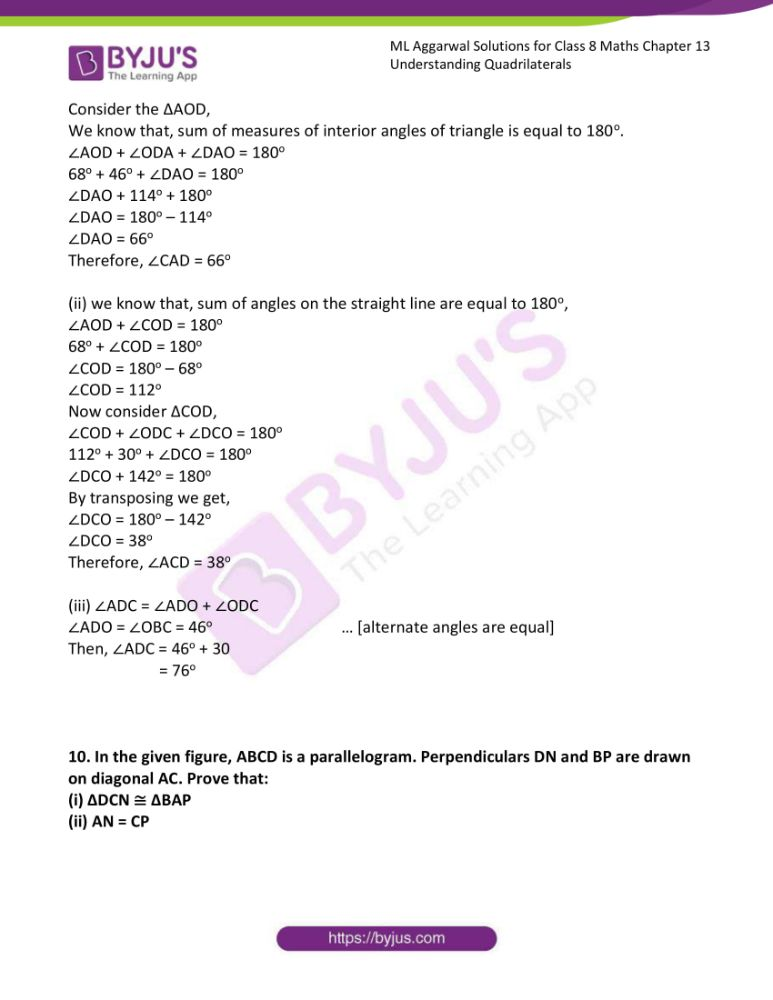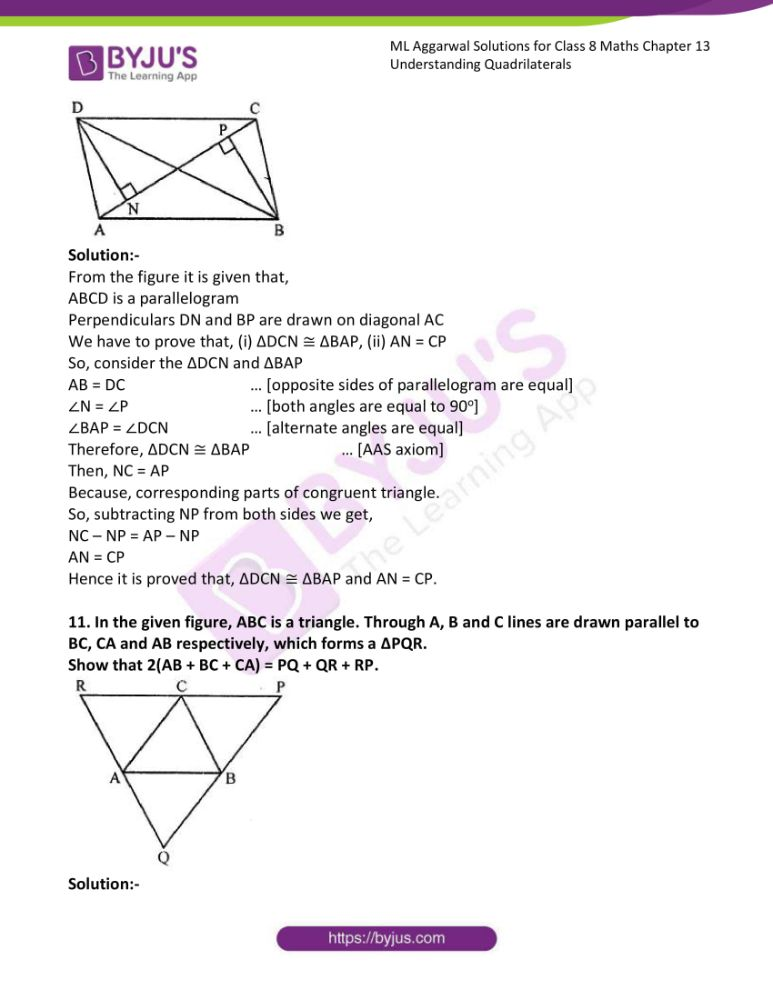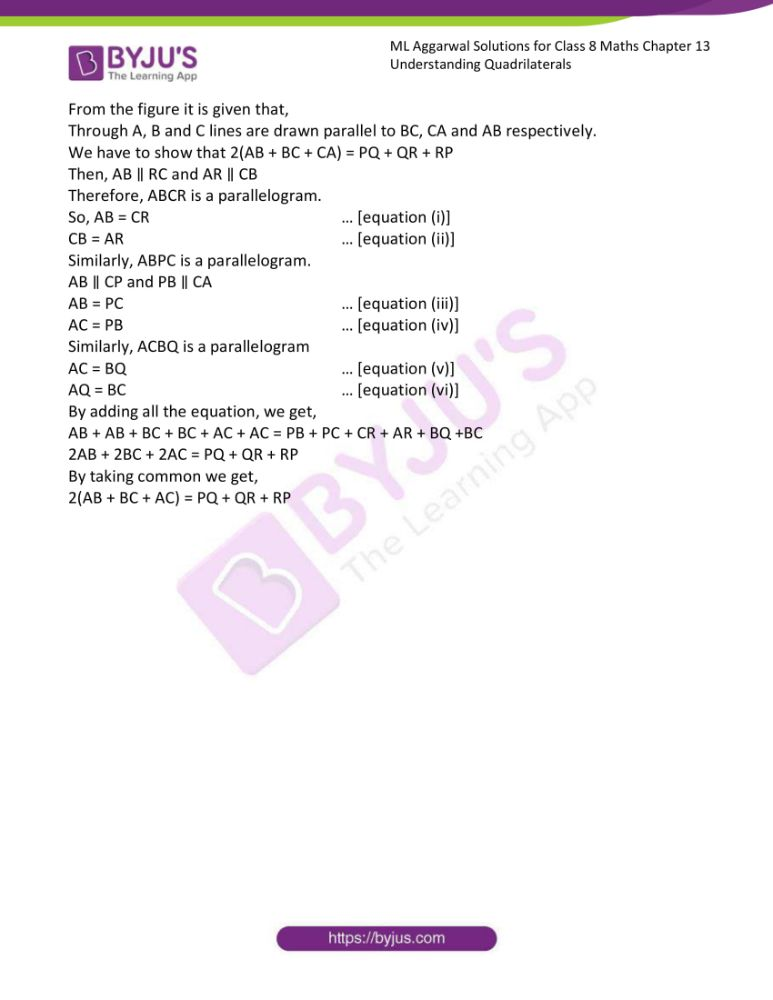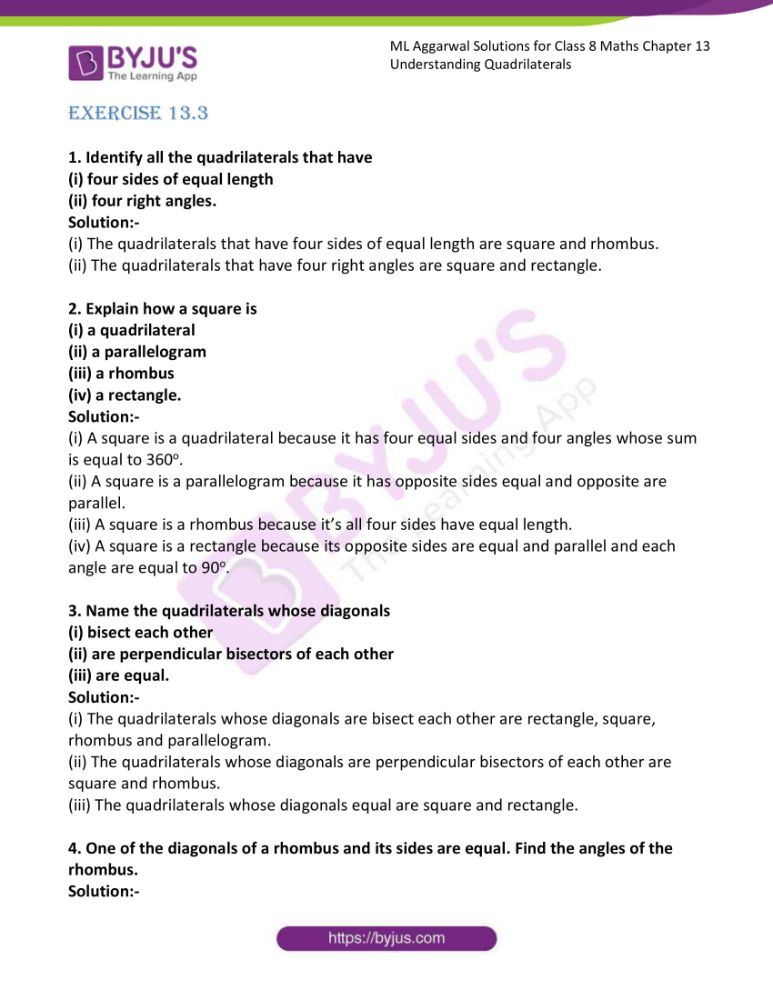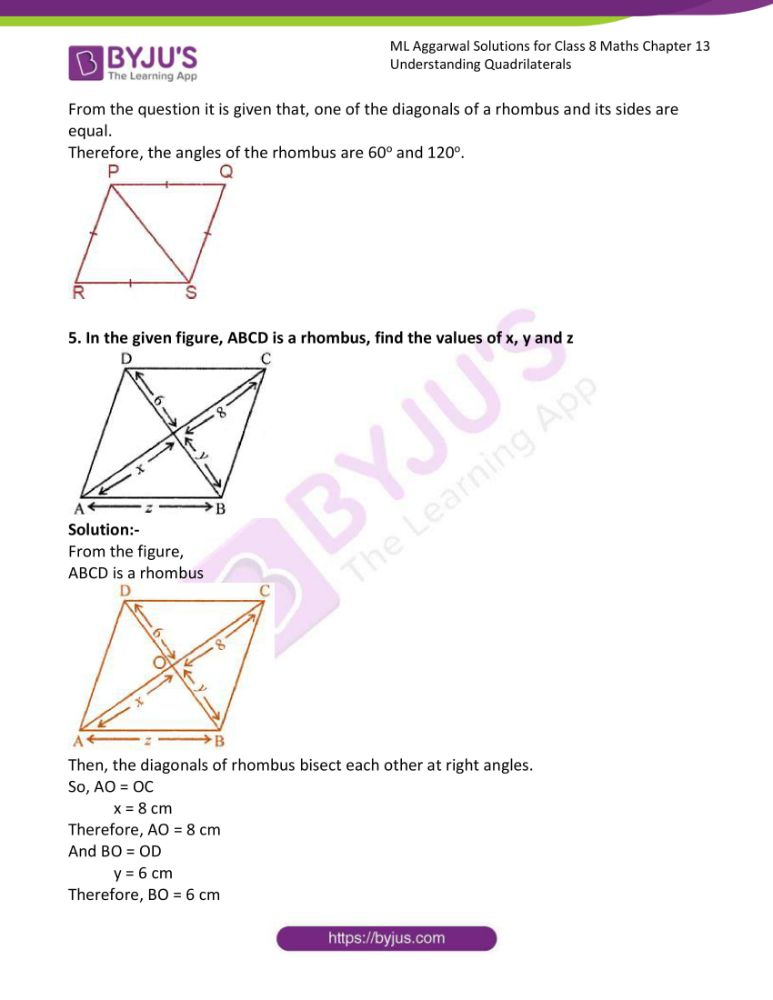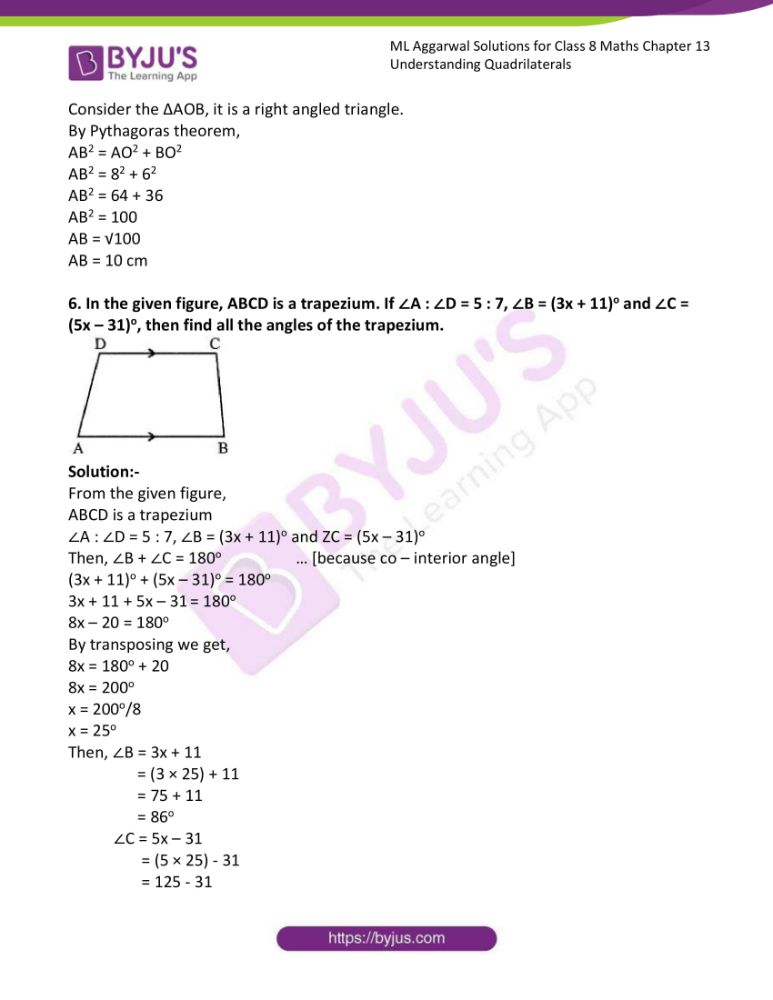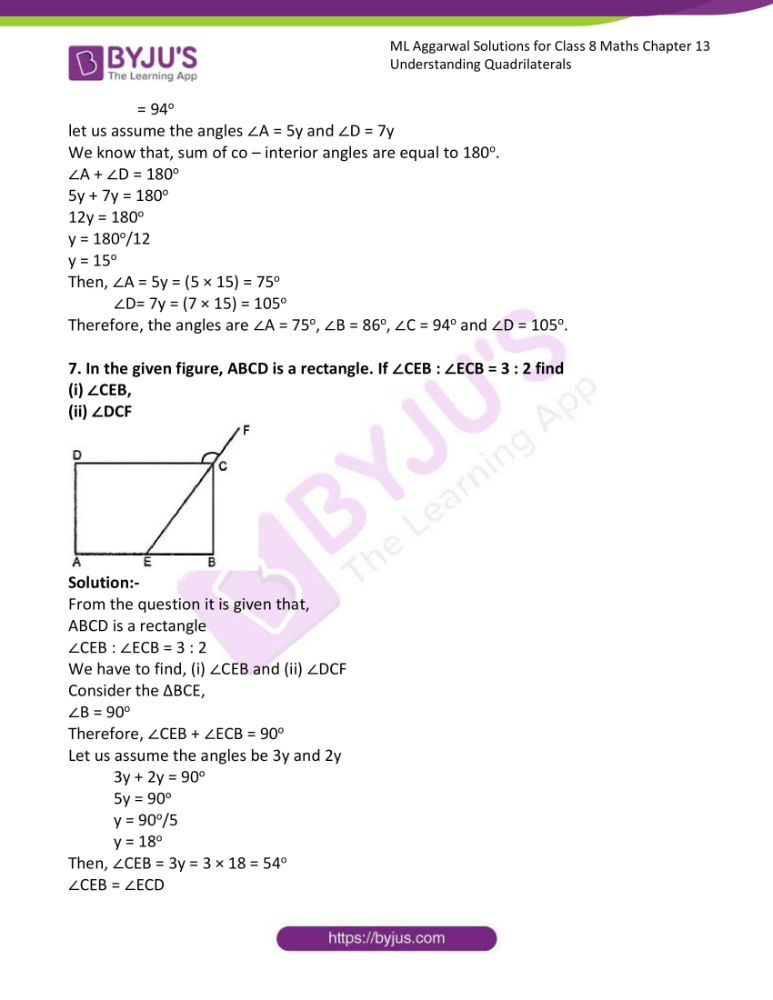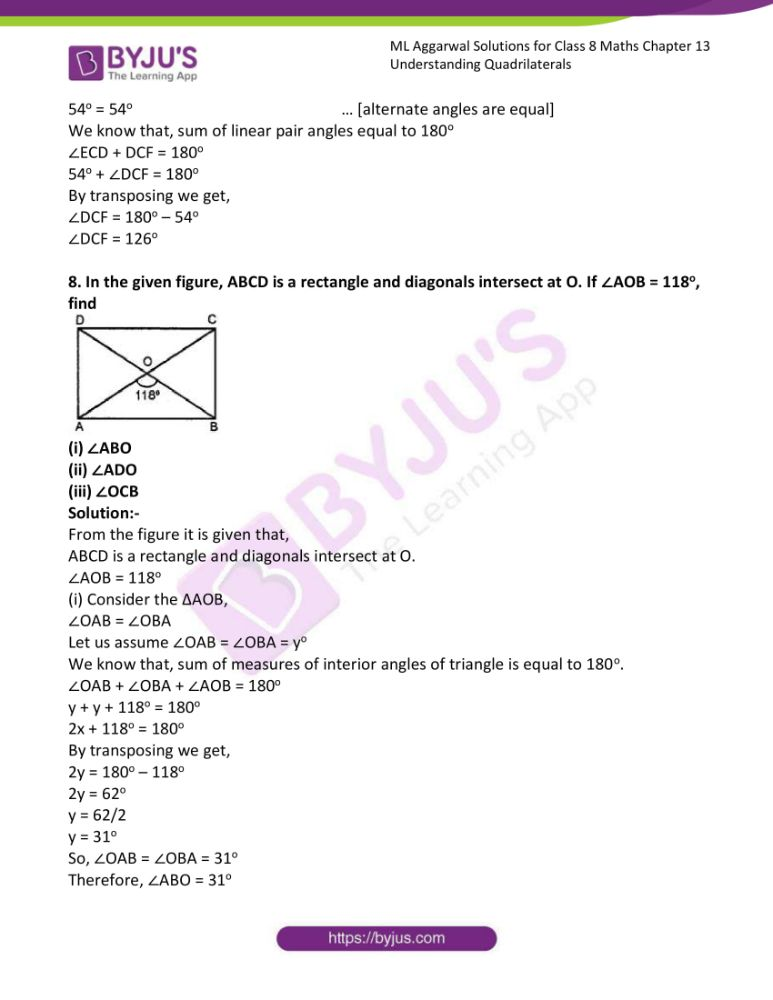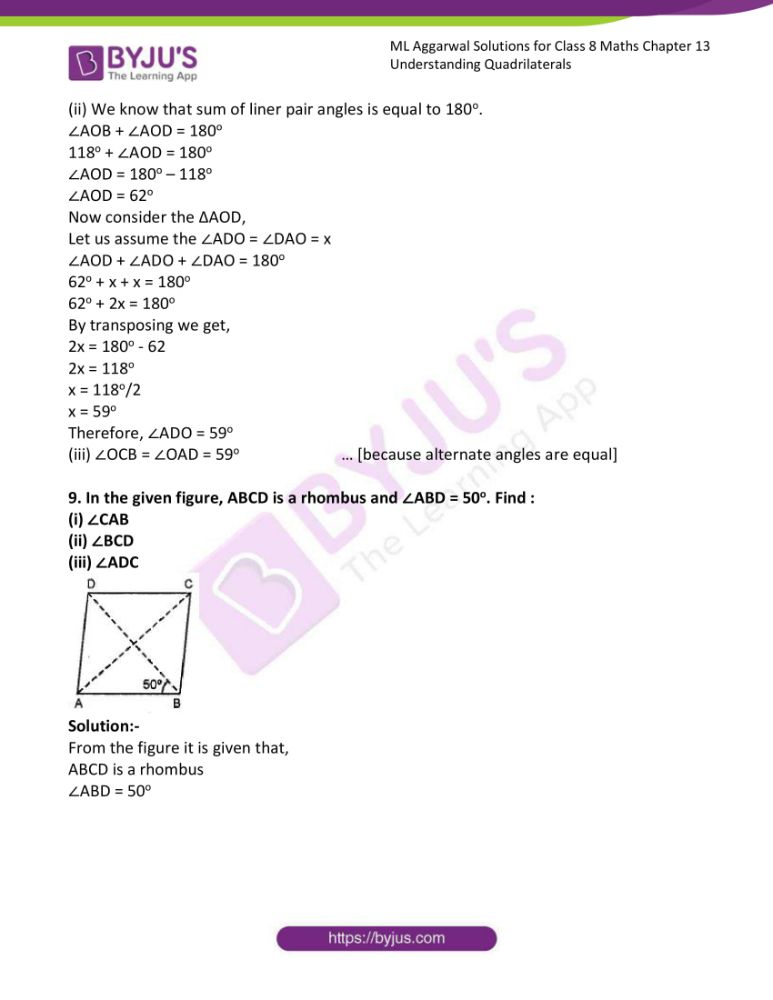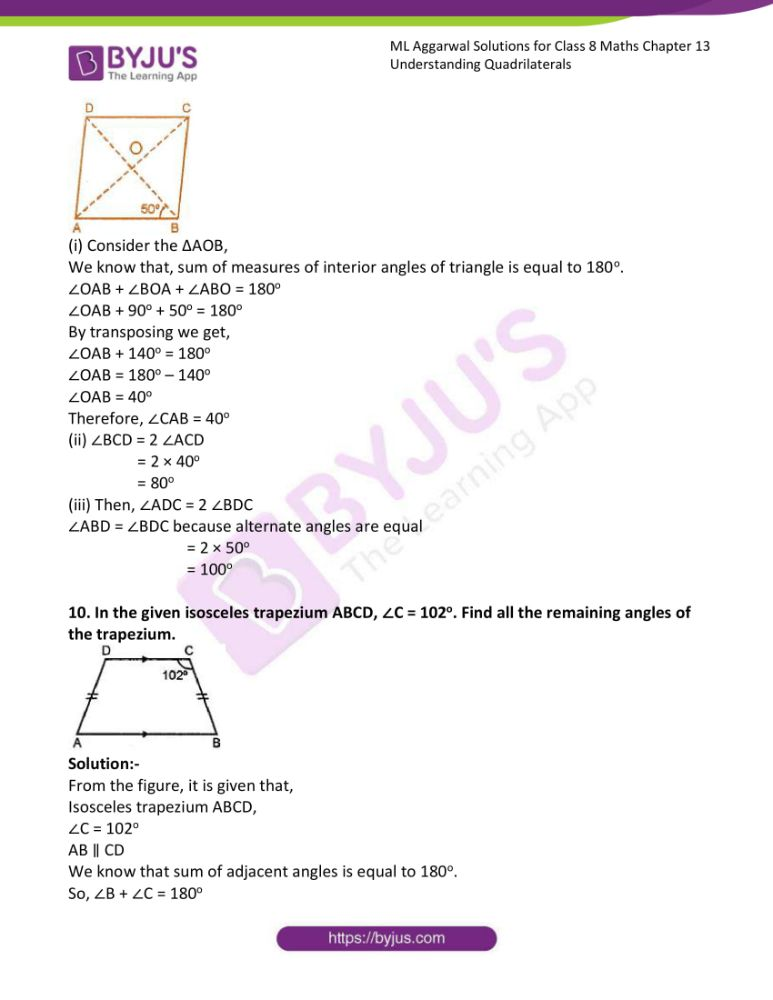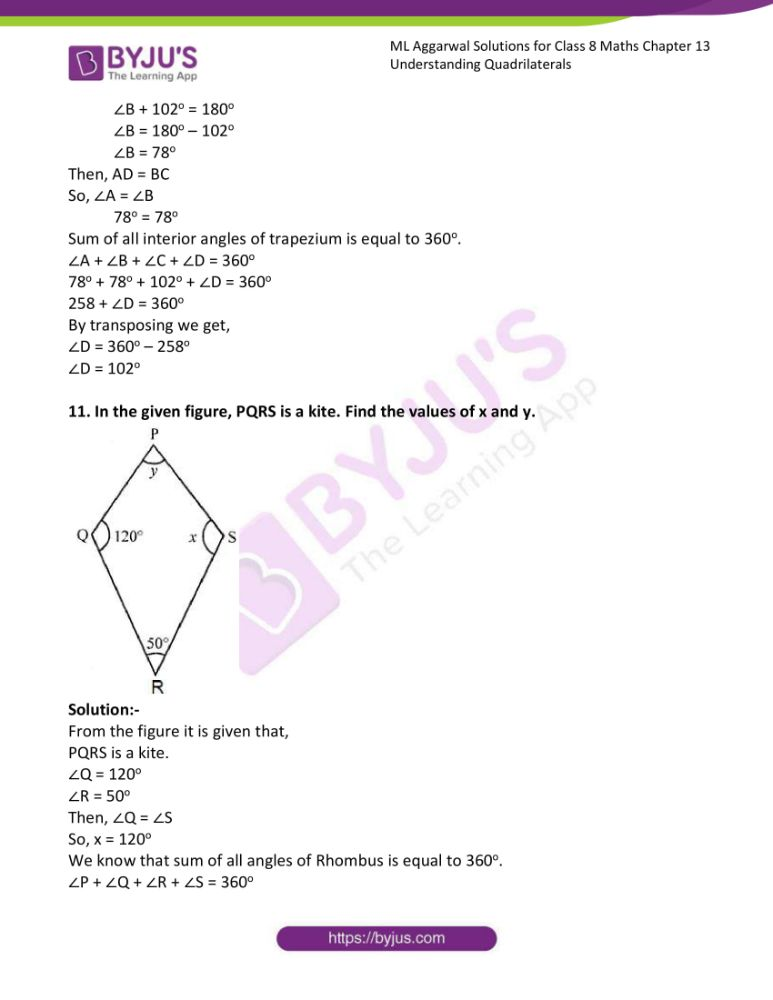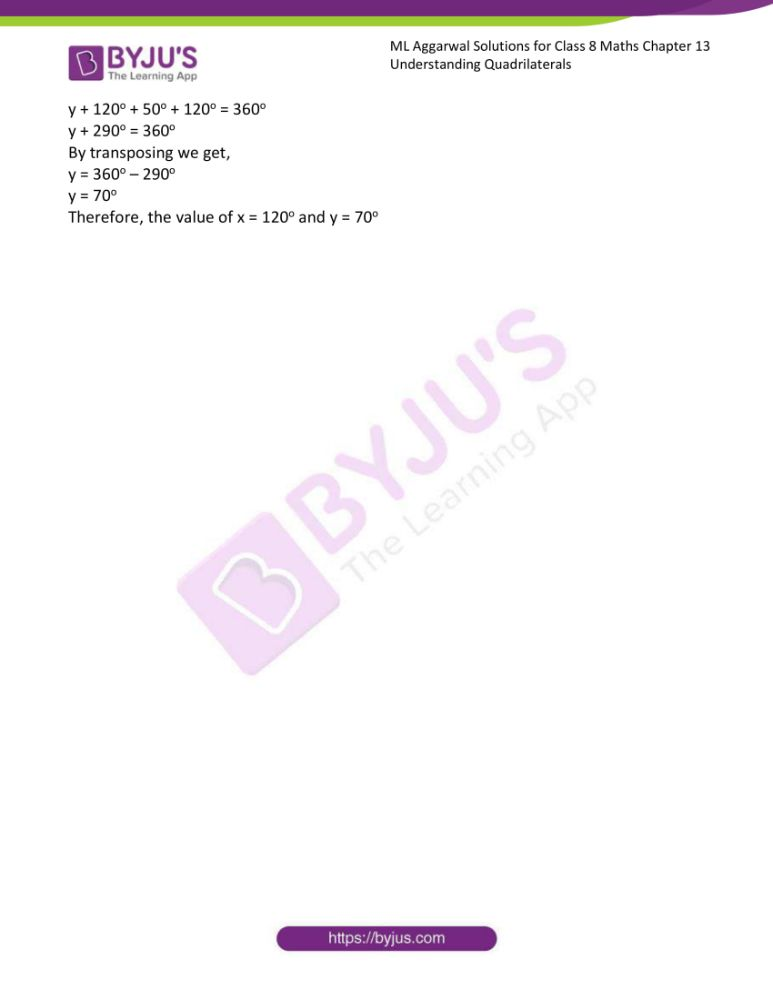### Access answers to ML Aggarwal Solutions for Class 8 Maths Chapter 13 Understanding Quadrilaterals

Exercise 13.1

1. Some figures are given below.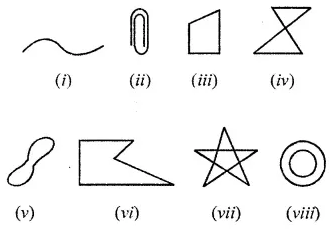Classify each of them on the basis of the following:
(a) Simple curve
(b) Simple closed curve
(c) Polygon
(d) Convex polygon
(e) Concave polygon

Solution:-

The given figure are classified as,

(a) Figure (i), Figure (ii), Figure (iii), Figure (v) and Figure (vi) are Simple curves.

Simple curve is a curve that does not cross itself.

(b) Figure (iii), Figure (v) and Figure (vi) are Simple closed curves.

In simple closed curves the shapes are closed by line-segments or by a curved line.

(c) Figure (iii) and Figure (vi) are Polygons.

A Polygon is any 2-dimensional shape formed with straight lines.

(d) Figure (iii) is a Convex polygon.

In a convex polygon, every diagonal of the figure passes only through interior points of the polygon.

(e) Figure (vi) is a Concave polygon.

In a concave polygon, at least one diagonal of the figure contains points that are exterior to the polygon.

2. How many diagonals does each of the following have?
(b) A regular hexagon
Solution:

(a) A convex quadrilateral has two diagonals.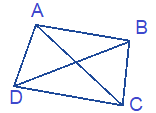(b) A regular hexagon has 9 diagonals as shown.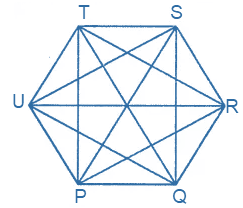3. Find the sum of measures of all interior angles of a polygon with the number of sides:
(i) 8
(ii) 12
Solution:

From the question it is given that,

(i) 8

We know that,

Sum of measures of all interior angles of 8 sided polygons = (2n – 4) × 90o

Where, n = 8

= ((2 × 8) – 4) × 90o

= (16 – 4) × 90o

= 12 × 90o

= 1080o

(ii) 12

We know that,

Sum of measures of all interior angles of 12 sided polygons = (2n – 4) × 90o

Where, n = 12

= ((2 × 12) – 4) × 90o

= (24 – 4) × 90o

= 20 × 90o

= 1800o

4. Find the number of sides of a regular polygon whose each exterior angles has a measure of
(i) 24o
(ii) 60o
(iii) 72o
Solution:-

(i) The number of sides of a regular polygon whose each exterior angles has a measure of 24o

Let us assume the number of sides of the regular polygon be n,

Then, n = 360o/24o

n = 15

Therefore, the number of sides of a regular polygon is 15.

(ii) The number of sides of a regular polygon whose each exterior angles has a measure of 60o

Let us assume the number of sides of the regular polygon be n,

Then, n = 360o/60o

n = 6

Therefore, the number of sides of a regular polygon is 6.

(iii) The number of sides of a regular polygon whose each exterior angles has a measure of 72o

Let us assume the number of sides of the regular polygon be n,

Then, n = 360o/72o

n = 5

Therefore, the number of sides of a regular polygon is 5.

5. Find the number of sides of a regular polygon if each of its interior angles is
(i) 90o
(ii) 108o
(iii) 165o
Solution:-

(i) The number of sides of a regular polygon whose each interior angles has a measure of 90o

Let us assume the number of sides of the regular polygon be n,

Then, we know that 90o = ((2n – 4)/n) × 90o

90o/90o = (2n – 4)/n

1 = (2n – 4)/n

2n – 4 = n

By transposing we get,

2n – n = 4

n = 4

Therefore, the number of sides of a regular polygon is 4.

So, it is a Square.

(ii) The number of sides of a regular polygon whose each interior angles has a measure of 108o

Let us assume the number of sides of the regular polygon be n,

Then, we know that 108o = ((2n – 4)/n) × 90o

108o/90o = (2n – 4)/n

6/5 = (2n – 4)/n

By cross multiplication,

5(2n – 4) = 6n

10n – 20 = 6n

By transposing we get,

10n – 6n = 20

4n = 20

n = 20/4

n = 5

Therefore, the number of sides of a regular polygon is 5.

So, it is a Pentagon.

(iii) The number of sides of a regular polygon whose each interior angles has a measure of 165o

Let us assume the number of sides of the regular polygon be n,

Then, we know that 165o = ((2n – 4)/n) × 90o

165o/90o = (2n – 4)/n

11/6 = (2n – 4)/n

By cross multiplication,

6(2n – 4) = 11n

12n – 24 = 11n

By transposing we get,

12n – 11n = 24

n = 24

Therefore, the number of sides of a regular polygon is 24.

6. Find the number of sides in a polygon if the sum of its interior angles is:
(i) 1260o
(ii) 1980o
(iii) 3420o
Solution:-

(i) We know that,

Sum of measures of all interior angles of polygons = (2n – 4) × 90o

Given, interior angle = 1260o

1260 = (2n – 4) × 90o

1260/90 = 2n – 4

14 = 2n – 4

By transposing we get,

2n = 14 + 4

2n = 18

n = 18/2

n = 9

Therefore, the number of sides in a polygon is 9.

(ii) We know that,

Sum of measures of all interior angles of polygons = (2n – 4) × 90o

Given, interior angle = 1980o

1980 = (2n – 4) × 90o

1980/90 = 2n – 4

22 = 2n – 4

By transposing we get,

2n = 22 + 4

2n = 26

n = 26/2

n = 13

Therefore, the number of sides in a polygon is 13.

(ii) We know that,

Sum of measures of all interior angles of polygons = (2n – 4) × 90o

Given, interior angle = 3420o

3420 = (2n – 4) × 90o

3420/90 = 2n – 4

38 = 2n – 4

By transposing we get,

2n = 38 + 4

2n = 42

n = 42/2

n = 21

Therefore, the number of sides in a polygon is 21.

7. If the angles of a pentagon are in the ratio 7 : 8 : 11 : 13 : 15, find the angles.
Solution:-

From the question it is given that,

The angles of a pentagon are in the ratio 7 : 8 : 11 : 13 : 15

We know that, Sum of measures of all interior angles of polygons = (2n – 4) × 90o

Given, n = 5

= ((2 × 5) – 4) × 90o

= (10 – 4) × 90o

= 6 × 90o

= 540o

Let us assume the angles of the pentagon be 7a, 8a, 11a, 13a and 15a.

Then, 7a + 8a + 11a + 13a + 15a = 540o

54a = 540o

a = 540/54

a = 10o

Therefore, the angles are 7a = 7 × 10 = 70o

8a = 8 × 10 = 80o

11a = 11 × 10 = 110o

13a = 13 × 10 = 130o

15a = 15 × 10 = 150o

8. The angles of a pentagon are xo, (x – 10)o, (x + 20)o, (2x – 44)o and (2x – 70)o Calculate x.
Solution:-

From the question it is given that, angles of a pentagon are xo, (x – 10)o, (x + 20)o, (2x – 44)o and (2x – 70)o

We know that, Sum of measures of all interior angles of polygons = (2n – 4) × 90o

Where, n = 5

= ((2 × 5) – 4) × 90o

= (10 – 4) × 90o

= 6 × 90o

= 540o

Then, xo+ (x – 10)o + (x + 20)o + (2x – 44)o + (2x – 70)o = 540

x + x – 10o + x + 20o + 2x – 44o + 2x – 70o = 540o

7x + 20o – 124o = 540o

7x – 104o = 540o

By transposing we get,

7x = 540o + 104o

7x = 644o

x = 644o/7

x = 92o

Therefore, the value of x is 92o.

9. The exterior angles of a pentagon are in ratio 1 : 2 : 3 : 4 : 5. Find all the interior angles of the pentagon.
Solution:-

From the question it is given that, the exterior angles of a pentagon are in ratio 1 : 2 : 3 : 4 : 5.

We know that, sum of exterior angles of pentagon is equal to 360o.

So, let us assume the angles of the pentagon be 1a, 2a, 3a, 4a and 5a.

1a + 2a + 3a + 4a + 5a = 360o

15a = 360o

a = 360o/15

a = 24o

Therefore, the angles of pentagon are, 1a = 1 × 24 = 24o

2a = 2 × 24 = 48o

3a = 3 × 24 = 72o

4a = 4 × 24 = 96o

5a = 5 × 24 = 120o

Then, interior angles of the pentagon are, 180o – 24o = 156o

180o – 48o = 132o

180o – 72o = 108o

180o – 96o = 84o

180o – 120o = 60o

10. In a quadrilateral ABCD, AB || DC. If ∠A : ∠D = 2:3 and ∠B : ∠C = ∠7 : 8, find the measure of each angle.
Solution:-

From the question it is given that,

In a quadrilateral ABCD, AB || DC. If ∠A : ∠D = 2:3 and ∠B : ∠C = ∠7 : 8,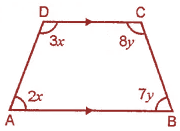Then, ∠A + ∠D = 180o

Let us assume the angle ∠A = 2a and ∠D = 3a

2a + 3a = 180o

5a = 180o

a = 180o/5

a = 36o

Therefore, ∠A = 2a = 2 × 36o = 72o

∠D = 3a = 3 × 36o = 108o

Now, ∠B + ∠C = 180o

Let us assume the angle ∠B = 7b and ∠C = 8b

7b + 8b = 180o

15b = 180o

b = 180o/15

b = 12o

Therefore, ∠B = 7b = 7 × 12o = 84o

∠C = 8b = 8 × 12o = 96o

11. From the adjoining figure, find
(i) x
(ii) ∠DAB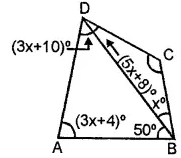Solution:-

(i) From the given figure,

∠A + ∠B + ∠C + ∠D = 360o

(3x + 4) + (50 + x) + (5x + 8) + (3x + 10) = 360o

3x + 4 + 50 + x + 5x + 8 + 3x + 20 = 360o

12x + 72 = 360o

By transposing we get,

12x = 360o – 72

12x = 288

x = 288/12

x = 24

(ii) ∠DAB = (3x + 4)

= ((3 × 24) + 4)

= 72 + 4

= 76o

Therefore, ∠DAB = 76o

(iii) Consider the triangle ABD,

We know that, sum of interior angles of triangle is equal to 180o,

∠DAB + ∠ABD + ∠ADB = 180o

76o + 50o + ∠ADB = 180o

12. Find the angle measure x in the following figures:

(i)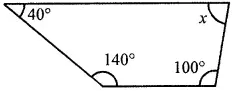Solution:-

From the given quadrilateral three angles are 40o, 100o and 140o

We have to find the value of x,

We know that, sum of four angles of quadrilateral is equal to 360o.

So, 40o + 100o + 140o + x = 360o

280o + x = 360o

x = 360o – 280o

x = 80o

Therefore, the value of x is 80o.

(ii)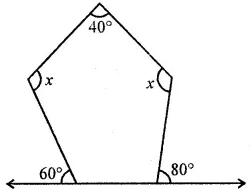Solution:-

From the given figure,

Let MNOPQ is a pentagon,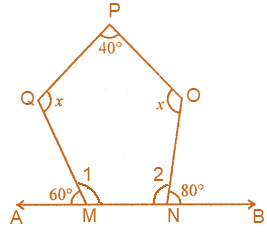We know that, sum of angles linear pair is equal to 180o

So, ∠1 + 60o = 180o

∠1 = 180o – 60o

∠1 = 120o

And ∠2 + 80o = 180o

∠2 = 180o – 80o

∠2 = 100o

Also We know that, Sum of measures of all interior angles of polygons = (2n – 4) × 90o

Where, n = 5

= ((2 × 5) – 4) × 90o

= (10 – 4) × 90o

= 6 × 90o

= 540o

Then, ∠M + ∠N + ∠O + ∠Q + ∠P = 540o

120o + 100o + x + x + 40o = 540o

260o + 2x = 540o

By transposing we get,

2x = 540o – 260o

2x = 280o

x = 280o/2

x = 140o

Therefore, the value of x is 140o.

(iii)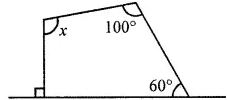Solution:-

From the given quadrilateral angles are 60o and 100o,

We know that, sum of angles linear pair is equal to 180o

So, another angle is 180o – 90o = 90o

We have to find the value of x,

We know that, sum of four angles of quadrilateral is equal to 360o.

So, 60o + 100o + 90o + x = 360o

250o + x = 360o

x = 360o – 250o

x = 110o

Therefore, the value of x is 110o.

(iv)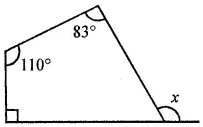Solution:-

We know that, sum of four angles of quadrilateral is equal to 360o.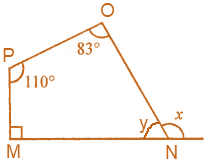∠M + ∠N + ∠O + ∠P = 360o

90o + y + 83o + 110o = 360o

283o + y = 360o

y = 360o – 283o

y = 77o

Therefore, the value of y is 110o

We know that, sum of angles linear pair is equal to 180o

So, y + x = 180o

77o + x = 180o

By transposing we get,

x = 180o – 77o

x = 103o

Therefore, the value of x 103o.

13.

(i) In the given figure, find x + y + z.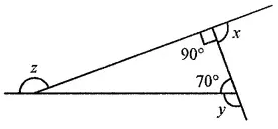Solution:-

From the figure,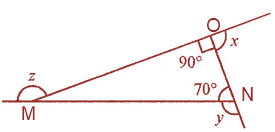Consider the triangle MNO,

We know that, sum of measures of interior angles of triangle is equal to 180o.

∠M + ∠N + ∠O = 180o

∠M + 70o + 90o = 180o

160o + ∠M = 180o

∠M = 180o – 160

∠M = 20o

We know that, sum of angles linear pair is equal to 180o

So, x + 90 = 180o

By transposing we get,

x = 180o – 90o

x = 90o

Therefore, the value of x is 90o.

Then, y + 70o = 180o

By transposing we get,

y = 180o – 70o

y = 110o

Therefore, the value of y is 110o.

Similarly, z + 20 = 180o

By transposing we get,

z = 180o – 20o

z = 160o

Therefore, the value of z is 160o.

Hence, x + y + z

= 90o + 110o + 160o

= 360o

(ii) In the given figure, find x + y + z + w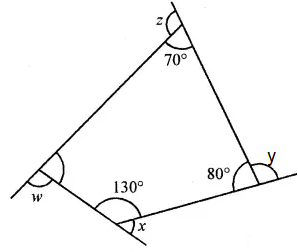Solution:-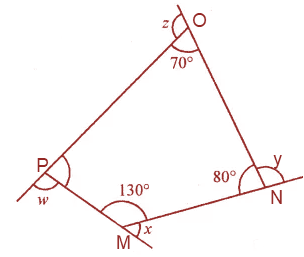We know that, sum of four angles of quadrilateral is equal to 360o.

∠M + ∠N + ∠O + ∠P = 360o

130o + 80o + 70o + ∠P = 360o

280o + ∠P = 360o

∠P = 360o – 280o

∠P = 80o

We know that, sum of angles linear pair is equal to 180o

So, x + 130o = 180o

By transposing we get,

x = 180o – 130o

x = 50o

Therefore, the value of x is 50o.

Then, y + 80o = 180o

By transposing we get,

y = 180o – 80o

y = 100o

Therefore, the value of y is 100o.

Similarly, z + 70 = 180o

By transposing we get,

z = 180o – 70o

z = 110o

Therefore, the value of z is 110o.

Similarly, w + 80 = 180o

By transposing we get,

z = 180o – 80o

z = 100o

Therefore, the value of z is 110o.

Hence, x + y + z + w

= 50o + 100o + 110o + 100

= 360o

14. A heptagon has three equal angles each of 120o and four equal angles. Find the size of equal angles.
Solution:-

From the question it is given that,

A heptagon has three equal angles each of 120o

Four equal angles = ?

We know that, Sum of measures of all interior angles of polygons = (2n – 4) × 90o

Where, n = 7

= ((2 × 7) – 4) × 90o

= (14 – 4) × 90o

= 10 × 90o

= 900o

Sum of 3 equal angles = 120o + 120o + 120o = 360o

Let us assume the sum of four equal angle be 4x,

So, sum of 7 angles of heptagon = 900o

Sum of 3 equal angles + Sum of 4 equal angles = 900o

360o + 4x = 900o

By transposing we get,

4x = 900o – 360o

4x = 540o

x = 540o/4

x = 134o

Therefore, remaining four equal angle measures 135o each.

15. The ratio between an exterior angle and the interior angle of a regular polygon is 1 : 5. Find
(i) the measure of each exterior angle
(ii) the measure of each interior angle
(iii) the number of sides in the polygon.

Solution:-

From the question it is given that,

The ratio between an exterior angle and the interior angle of a regular polygon is 1: 5

Let us assume exterior angle be y

And interior angle be 5y

We know that, sum of interior and exterior angle is equal to 180o,

y + 5y = 180o

6y = 180o

y = 180o/6

y = 30o

(i) the measure of each exterior angle = y = 30o

(ii) the measure of each interior angle = 5y = 5 × 30o = 150o

(iii) the number of sides in the polygon

The number of sides of a regular polygon whose each interior angles has a measure of 150o

Let us assume the number of sides of the regular polygon be n,

Then, we know that 150o = ((2n – 4)/n) × 90o

150o/90o = (2n – 4)/n

5/3 = (2n – 4)/n

By cross multiplication,

3(2n – 4) = 5n

6n – 12 = 5n

By transposing we get,

6n – 5n = 12

n = 12

Therefore, the number of sides of a regular polygon is 12.

16. Each interior angle of a regular polygon is double of its exterior angle. Find the number of sides in the polygon.

Solution:-

From the question it is given that,

Each interior angle of a regular polygon is double of its exterior angle.

So, let us assume exterior angle be y

Interior angle be 2y,

We know that, sum of interior and exterior angle is equal to 180o,

y + 2y = 180o

3y = 180o

y = 180o/3

y = 60o

Then, interior angle = 2y = 2 × 60o = 120o

The number of sides of a regular polygon whose each interior angles has a measure of 120o

Let us assume the number of sides of the regular polygon be n,

Then, we know that 120o = ((2n – 4)/n) × 90o

120o/90o = (2n – 4)/n

4/3 = (2n – 4)/n

By cross multiplication,

3(2n – 4) = 4n

6n – 12 = 4n

By transposing we get,

6n – 4n = 12

2n = 12

n = 12/2

n = 6

Therefore, the number of sides of a regular polygon is 6.

Exercise 13.2

1. In the given figure, ABCD is a parallelogram. Complete each statement along with the definition or property used.
(ii) DC = ………..
(iii) ∠DCB = ………..
(v) ∠DAB = ………..
(vi) OC = ………..
(vii) OB = ………..
(viii) m∠DAB + m∠CDA = ………..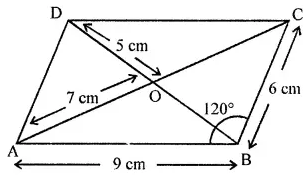Solution:-

From the given figure,

(i) AD = 6 cm … [because opposite sides of parallelogram are equal]

(ii) DC = 9 cm … [because opposite sides of parallelogram are equal]

(iii) ∠DCB = 60o

(iv) ∠ADC = ∠ABC = 120o

(v) ∠DAB = ∠DCB = 60o

(vi) OC = AO = 7 cm

(vii) OB = OD = 5 cm

(viii) m∠DAB + m∠CDA = 180o

2. Consider the following parallelograms. Find the values of x, y, z in each.

(i)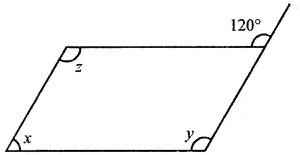Solution:-

(i) Consider parallelogram MNOP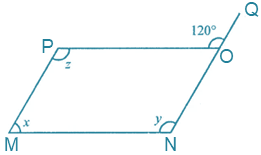From the figure, ∠POQ = 120o

We know that, sum of angles linear pair is equal to 180o

So, ∠POQ + ∠PON = 180o

120o + ∠PON = 180o

∠PON = 180o – 120o

∠PON = 60o

Then, ∠M = ∠O = 60o … [because opposite angles of parallelogram are equal]

∠POQ = ∠MNO

120o = 120o … [because corresponding angels are equal]

Hence, y = 120o

Also, z = y

120o = 120o

Therefore, x = 60o, y = 120o and z = 120o

(ii)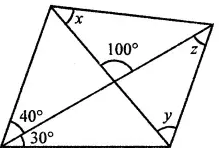Solution:-

Solution:-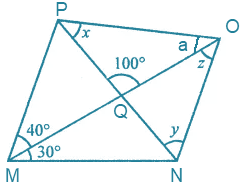From the figure, it is given that ∠PQO = 100o, ∠OMN = 30o, ∠PMO =40o.

Then, ∠NOM = ∠OMP … [because alternate angles are equal]

So, z = 40o

Now, ∠NMO = ∠POM … [because alternate angles are equal]

So, ∠NMO = a = 30o

Consider the triangle PQO,

We know that, sum of measures of interior angles of triangle is equal to 180o.

∠P + ∠Q + ∠O = 180o

x + 100o + 30o = 180o

x + 130o = 180o

x = 180o – 130o

x = 50o

Then, exterior angle ∠OQP = y + z

100o = y + 40o

By transposing we get,

y = 100o – 40o

y = 60o

Therefore, the value of x = 50o, y = 60o and z = 40o.

(iii)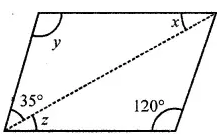Solution:-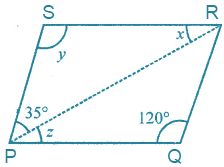From the above figure,

∠SPR = ∠PRQ

35o = 35o … [because alternate angles are equal]

Now consider the triangle PQR,

We know that, sum of measures of interior angles of triangle is equal to 180o.

∠RPQ + ∠PQR + ∠PRQ = 180o

z + 120o + 35o = 180o

z + 155o = 180o

z = 180o – 155o

z = 25o

Then, ∠QPR = ∠PRQ

Z = x

25o = 25o … [because alternate angles are equal]

We know that, in parallelogram opposite angles are equal.

So, ∠S = ∠Q

y = 120o

Therefore, value of x = 25o, y = 120o and ∠z = 25o.

(iv)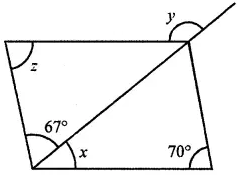Solution:-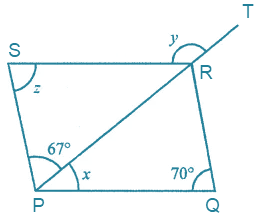From the above figure, it is given that ∠SPR = 67o and ∠PQR = 70o

∠SPR = ∠PRQ

67o = 67o … [because alternate angles are equal]

Now, consider the triangle PQR

We know that, sum of measures of interior angles of triangle is equal to 180o.

∠RPQ + ∠PQR + ∠PRQ = 180o

x + 70o + 67o = 180o

x + 137o = 180o

x = 180o – 137o

x = 43o

Then, ∠PSR = ∠PQR

We know that, in parallelogram opposite angles are equal.

Z = 70o

Also we know that, exterior angle ∠SRT = ∠PSR + ∠SPR

y = 70o + 67o

y = 137o

Therefore, value of x = 43o, y = 137o and z = 70o

3. Two adjacent sides of a parallelogram are in the ratio 5 : 7. If the perimeter of a parallelogram is 72 cm, find the length of its sides.
Solution:-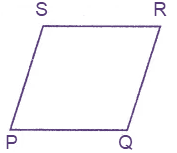Consider the parallelogram PQRS,

From the question it is given that, two adjacent sides of a parallelogram are in the ratio 5 : 7.

Perimeter of parallelogram = 72 cm

2(SP + RQ) = 72 cm

SP + RQ = 72/2

SP + RQ = 36 cm

Let us assume the length of side SP = 5y and RQ = 7y,

5y + 7y = 36

12y = 36

y = 36/12

y = 3

Therefore, SP = 5y = 5 × 3 = 15 cm

RQ = 7y = 7 × 3 = 21 cm

4. The measure of two adjacent angles of a parallelogram is in the ratio 4 : 5. Find the measure of each angle of the parallelogram.
Solution:-Consider the parallelogram PQRS,

From the question it is given that, The measure of two adjacent angles of a parallelogram is in the ratio 4 : 5.

So, ∠P: ∠Q = 4: 5

Let us assume the ∠P = 4y and ∠Q = 5y.

Then, we know that, ∠P + ∠Q = 180o

4y + 5y = 180o

9y = 180o

y = 180o/9

y = 20o

Therefore, ∠P = 4y = 4 × 20o = 80o and ∠Q = 5y = (5 × 20o) = 100o

In parallelogram opposite angles are equal,

So, ∠R = ∠P = 80o

∠S = ∠Q = 100o

(i) ∠A + ∠C= 180o?
(ii) AD = BC = 6 cm, AB = 5 cm, DC = 4.5 cm?
(iii) ∠B = 80o, ∠D = 70o?
(iv) ∠B + ∠C= 180o?

Solution:-

From the question it is given that, quadrilateral ABCD can be a parallelogram.

We know that in parallelogram opposite sides are equal and opposites angles are equal.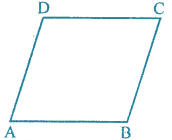So, AB = DC and AD = BC also ∠A = ∠C and ∠B = ∠D.

(i) ∠A + ∠C= 180o

From the above condition it may be a parallelogram and may not be a parallelogram.

(ii) AD = BC = 6 cm, AB = 5 cm, DC = 4.5 cm

From the above dimension not able to form parallelogram.

Because AB ≠ DC

(iii) ∠B = 80o, ∠D = 70o

From the above dimension not able to form parallelogram.

Because ∠B ≠ ∠D

(iv) ∠B + ∠C= 180o

From the above condition it may be a parallelogram and may not be a parallelogram.

6. In the following figures, HOPE and ROPE are parallelograms. Find the measures of angles x, y and z. State the properties you use to find them.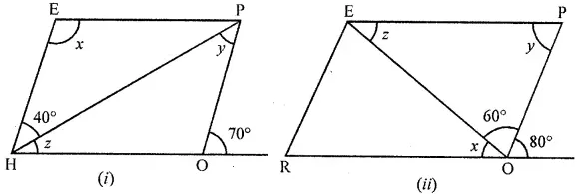Solution:

(i) Consider the parallelogram HOPE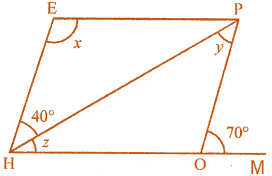We know that, sum of interior and exterior angle is equal to 180o,

∠HOP + ∠POM = 180o

∠HOP + 70o = 180o – 70o

∠HOP = 110o

Then, ∠HEP = ∠HOP

x = 110o … [because in parallelogram opposite angles are equal]

∠OPH = ∠PHE

y = 40o … [because alternate angles are equal]

Now, consider the triangle HOP

We know that, sum of measures of interior angles of triangle is equal to 180o.

∠PHO + ∠HOP + ∠OPH = 180o

z + 110o + 40o = 180o

z + 150o = 180o

z = 180o – 150o

z = 30o

Therefore, value of x = 110o, y = 40o and z = 30o.

(ii) Consider the parallelogram ROPE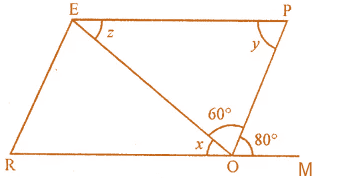From the figure, it is given that ∠POM = 80o and ∠POE = 60o.

Then, ∠OPE = ∠POM

y = 80o … [because alternate angles are equal]

We know that, angles on the same straight line are equal to 180o.

∠ROE + ∠EOP + POM = 180o

x + 60o + 80o = 180o

x + 140o = 180o

By transposing we get,

x = 180o – 140o

x = 40o

Then, ∠ROE = ∠OEP

x = z

40o = z

Therefore, value of x = 40o, y = 80o and z = 40o.

7. In the given figure TURN and BURN are parallelograms. Find the measures of x and y (lengths are in cm).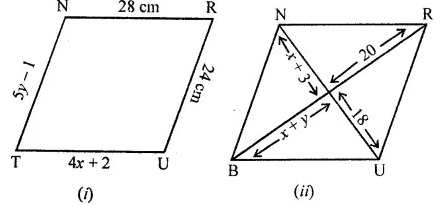Solution:-

(i) Consider the parallelogram TURN

We know that, in parallelogram opposite sides are equal.

So, TU = RN

4x + 2 = 28

By transposing,

4x = 28 – 2

4x = 26

x = 26/4

x = 6.5 cm

and NT = RU

5y – 1 = 24

5y = 24 + 1

5y = 25

y = 25/5

y = 5

Therefore, value of x = 6.5 cm and y = 5 cm.

(ii) Consider the parallelogram BURN,

BO = OR

x + y = 20 … [equation (i)]

UO = ON

x + 3 = 18

x = 18 – 3

x = 15

substitute the value of x in equation (i),

15 + y = 20

y = 20 – 15

y = 5

Therefore, value of x = 15 and y = 5.

8. In the following figure, both ABCD and PQRS are parallelograms. Find the value of x.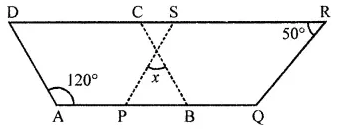Solution:-

From the figure it is given that, ABCD and PQRS are two parallelograms.

∠A = 120o and ∠R = 50o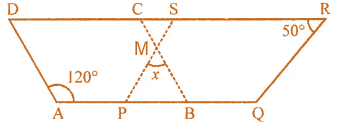We know that, ∠A + ∠B = 180o

120o + ∠B = 180o

∠B = 180o – 120o

∠B = 60o

In parallelogram opposite angles are equal,

Then, consider the triangle MPB

We know that, sum of measures of interior angles of triangle is equal to 180o.

∠PMB + ∠P + ∠B = 180o

x + 50o + 60o = 180o

x + 110o = 180o

By transposing we get,

x = 180o – 110o

Therefore, value of x = 70o

9. In the given figure, ABCD, is a parallelogram and diagonals intersect at O. Find :
(ii) ∠ACD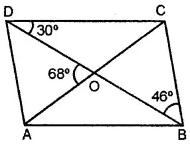Solution:-

From the figure it is given that,

∠CBD = 46o, ∠AOD = 68o and ∠BDC = 30o

(i) ∠CBD = ∠BDA = 46o … [alternate angles are equal]

Consider the ΔAOD,

We know that, sum of measures of interior angles of triangle is equal to 180o.

∠AOD + ∠ODA + ∠DAO = 180o

68o + 46o + ∠DAO = 180o

∠DAO + 114o + 180o

∠DAO = 180o – 114o

∠DAO = 66o

(ii) we know that, sum of angles on the straight line are equal to 180o,

∠AOD + ∠COD = 180o

68o + ∠COD = 180o

∠COD = 180o – 68o

∠COD = 112o

Now consider ΔCOD,

∠COD + ∠ODC + ∠DCO = 180o

112o + 30o + ∠DCO = 180o

∠DCO + 142o = 180o

By transposing we get,

∠DCO = 180o – 142o

∠DCO = 38o

Therefore, ∠ACD = 38o

∠ADO = ∠OBC = 46o … [alternate angles are equal]

Then, ∠ADC = 46o + 30

= 76o

10. In the given figure, ABCD is a parallelogram. Perpendiculars DN and BP are drawn on diagonal AC. Prove that:
(i) ∆DCN ≅ ∆BAP
(ii) AN = CP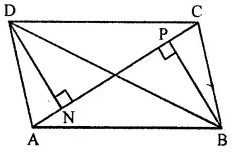Solution:-

From the figure it is given that,

ABCD is a parallelogram

Perpendiculars DN and BP are drawn on diagonal AC

We have to prove that, (i) ∆DCN ≅ ∆BAP, (ii) AN = CP

So, consider the ΔDCN and ΔBAP

AB = DC … [opposite sides of parallelogram are equal]

∠N = ∠P … [both angles are equal to 90o]

∠BAP = ∠DCN … [alternate angles are equal]

Therefore, ∆DCN ≅ ∆BAP … [AAS axiom]

Then, NC = AP

Because, corresponding parts of congruent triangle.

So, subtracting NP from both sides we get,

NC – NP = AP – NP

AN = CP

Hence it is proved that, ∆DCN ≅ ∆BAP and AN = CP.

11. In the given figure, ABC is a triangle. Through A, B and C lines are drawn parallel to BC, CA and AB respectively, which forms a ∆PQR.

Show that 2(AB + BC + CA) = PQ + QR + RP.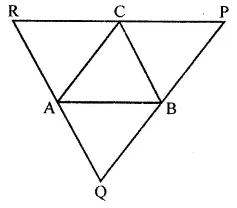Solution:-

From the figure it is given that,

Through A, B and C lines are drawn parallel to BC, CA and AB respectively.

We have to show that 2(AB + BC + CA) = PQ + QR + RP

Then, AB ∥ RC and AR ∥ CB

Therefore, ABCR is a parallelogram.

So, AB = CR … [equation (i)]

CB = AR … [equation (ii)]

Similarly, ABPC is a parallelogram.

AB ∥ CP and PB ∥ CA

AB = PC … [equation (iii)]

AC = PB … [equation (iv)]

Similarly, ACBQ is a parallelogram

AC = BQ … [equation (v)]

AQ = BC … [equation (vi)]

By adding all the equation, we get,

AB + AB + BC + BC + AC + AC = PB + PC + CR + AR + BQ +BC

2AB + 2BC + 2AC = PQ + QR + RP

By taking common we get,

2(AB + BC + AC) = PQ + QR + RP

Exercise 13.3

1. Identify all the quadrilaterals that have
(i) four sides of equal length
(ii) four right angles.

Solution:-

(i) The quadrilaterals that have four sides of equal length are square and rhombus.

(ii) The quadrilaterals that have four right angles are square and rectangle.

2. Explain how a square is
(ii) a parallelogram
(iii) a rhombus
(iv) a rectangle.

Solution:-

(i) A square is a quadrilateral because it has four equal sides and four angles whose sum is equal to 360o.

(ii) A square is a parallelogram because it has opposite sides equal and opposite are parallel.

(iii) A square is a rhombus because it’s all four sides have equal length.

(iv) A square is a rectangle because its opposite sides are equal and parallel and each angle are equal to 90o.

3. Name the quadrilaterals whose diagonals
(i) bisect each other
(ii) are perpendicular bisectors of each other
(iii) are equal.

Solution:-

(i) The quadrilaterals whose diagonals are bisect each other are rectangle, square, rhombus and parallelogram.

(ii) The quadrilaterals whose diagonals are perpendicular bisectors of each other are square and rhombus.

(iii) The quadrilaterals whose diagonals equal are square and rectangle.

4. One of the diagonals of a rhombus and its sides are equal. Find the angles of the rhombus.

Solution:-

From the question it is given that, one of the diagonals of a rhombus and its sides are equal.

Therefore, the angles of the rhombus are 60o and 120o.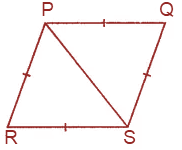5. In the given figure, ABCD is a rhombus, find the values of x, y and z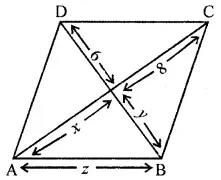Solution:-

From the figure,

ABCD is a rhombus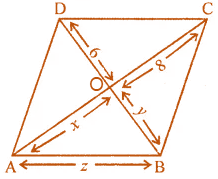Then, the diagonals of rhombus bisect each other at right angles.

So, AO = OC

x = 8 cm

Therefore, AO = 8 cm

And BO = OD

y = 6 cm

Therefore, BO = 6 cm

Consider the ∆AOB, it is a right angled triangle.

By Pythagoras theorem,

AB2 = AO2 + BO2

AB2 = 82 + 62

AB2 = 64 + 36

AB2 = 100

AB = √100

AB = 10 cm

6. In the given figure, ABCD is a trapezium. If ∠A : ∠D = 5 : 7, ∠B = (3x + 11)o and ∠C = (5x – 31)o, then find all the angles of the trapezium.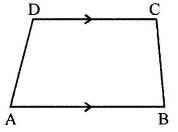Solution:-

From the given figure,

ABCD is a trapezium

∠A : ∠D = 5 : 7, ∠B = (3x + 11)o and ZC = (5x – 31)o

Then, ∠B + ∠C = 180o … [because co – interior angle]

(3x + 11)o + (5x – 31)o = 180o

3x + 11 + 5x – 31 = 180o

8x – 20 = 180o

By transposing we get,

8x = 180o + 20

8x = 200o

x = 200o/8

x = 25o

Then, ∠B = 3x + 11

= (3 × 25) + 11

= 75 + 11

= 86o

∠C = 5x – 31

= (5 × 25) – 31

= 125 – 31

= 94o

let us assume the angles ∠A = 5y and ∠D = 7y

We know that, sum of co – interior angles are equal to 180o.

∠A + ∠D = 180o

5y + 7y = 180o

12y = 180o

y = 180o/12

y = 15o

Then, ∠A = 5y = (5 × 15) = 75o

∠D= 7y = (7 × 15) = 105o

Therefore, the angles are ∠A = 75o, ∠B = 86o, ∠C = 94o and ∠D = 105o.

7. In the given figure, ABCD is a rectangle. If ∠CEB : ∠ECB = 3 : 2 find
(i) ∠CEB,
(ii) ∠DCF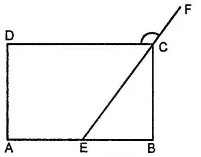Solution:-

From the question it is given that,

ABCD is a rectangle

∠CEB : ∠ECB = 3 : 2

We have to find, (i) ∠CEB and (ii) ∠DCF

Consider the ∆BCE,

∠B = 90o

Therefore, ∠CEB + ∠ECB = 90o

Let us assume the angles be 3y and 2y

3y + 2y = 90o

5y = 90o

y = 90o/5

y = 18o

Then, ∠CEB = 3y = 3 × 18 = 54o

∠CEB = ∠ECD

54o = 54o … [alternate angles are equal]

We know that, sum of linear pair angles equal to 180o

∠ECD + DCF = 180o

54o + ∠DCF = 180o

By transposing we get,

∠DCF = 180o – 54o

∠DCF = 126o

8. In the given figure, ABCD is a rectangle and diagonals intersect at O. If ∠AOB = 118o, find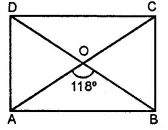(i) ∠ABO
(iii) ∠OCB

Solution:-

From the figure it is given that,

ABCD is a rectangle and diagonals intersect at O.

∠AOB = 118o

(i) Consider the ΔAOB,

∠OAB = ∠OBA

Let us assume ∠OAB = ∠OBA = yo

We know that, sum of measures of interior angles of triangle is equal to 180o.

∠OAB + ∠OBA + ∠AOB = 180o

y + y + 118o = 180o

2x + 118o = 180o

By transposing we get,

2y = 180o – 118o

2y = 62o

y = 62/2

y = 31o

So, ∠OAB = ∠OBA = 31o

Therefore, ∠ABO = 31o

(ii) We know that sum of liner pair angles is equal to 180o.

∠AOB + ∠AOD = 180o

118o + ∠AOD = 180o

∠AOD = 180o – 118o

∠AOD = 62o

Now consider the ΔAOD,

Let us assume the ∠ADO = ∠DAO = x

∠AOD + ∠ADO + ∠DAO = 180o

62o + x + x = 180o

62o + 2x = 180o

By transposing we get,

2x = 180o – 62

2x = 118o

x = 118o/2

x = 59o

(iii) ∠OCB = ∠OAD = 59o  … [because alternate angles are equal]

9. In the given figure, ABCD is a rhombus and ∠ABD = 50o. Find :
(i) ∠CAB
(ii) ∠BCD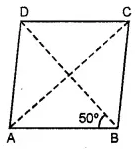Solution:-

From the figure it is given that,

ABCD is a rhombus

∠ABD = 50o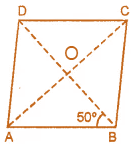(i) Consider the ΔAOB,

We know that, sum of measures of interior angles of triangle is equal to 180o.

∠OAB + ∠BOA + ∠ABO = 180o

∠OAB + 90o + 50o = 180o

By transposing we get,

∠OAB + 140o = 180o

∠OAB = 180o – 140o

∠OAB = 40o

Therefore, ∠CAB = 40o

(ii) ∠BCD = 2 ∠ACD

= 2 × 40o

= 80o

(iii) Then, ∠ADC = 2 ∠BDC

∠ABD = ∠BDC because alternate angles are equal

= 2 × 50o

= 100o

10. In the given isosceles trapezium ABCD, ∠C = 102o. Find all the remaining angles of the trapezium.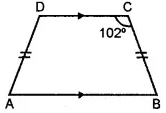Solution:-

From the figure, it is given that,

Isosceles trapezium ABCD,

∠C = 102o

AB ∥ CD

We know that sum of adjacent angles is equal to 180o.

So, ∠B + ∠C = 180o

∠B + 102o = 180o

∠B = 180o – 102o

∠B = 78o

So, ∠A = ∠B

78o = 78o

Sum of all interior angles of trapezium is equal to 360o.

∠A + ∠B + ∠C + ∠D = 360o

78o + 78o + 102o + ∠D = 360o

258 + ∠D = 360o

By transposing we get,

∠D = 360o – 258o

∠D = 102o

11. In the given figure, PQRS is a kite. Find the values of x and y.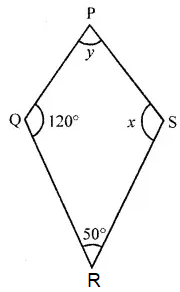Solution:-

From the figure it is given that,

PQRS is a kite.

∠Q = 120o

∠R = 50o

Then, ∠Q = ∠S

So, x = 120o

We know that sum of all angles of Rhombus is equal to 360o.

∠P + ∠Q + ∠R + ∠S = 360o

y + 120o + 50o + 120o = 360o

y + 290o = 360o

By transposing we get,

y = 360o – 290o

y = 70o

Therefore, the value of x = 120o and y = 70o